# PMean: Nonlinear regression for the difference of two exponentials

I wanted to provide an overview of how you analyze a classic nonlinear regression model. It is a difference of two exponential functions. This nonlinear function is used commonly in pharmocokinetic models and is a simply way to model the oral administration of a drug. I want to show how the model works in a mathematical sense and then how you fit it using R.

Here are some simple examples of nonlinear regression. We will use the built-in data set Theoph for all our examples.

``````# start without any extraneous variables
rm(list=ls())
``````##   Subject   Wt Dose Time  conc
## 1       1 79.6 4.02 0.00  0.74
## 2       1 79.6 4.02 0.25  2.84
## 3       1 79.6 4.02 0.57  6.57
## 4       1 79.6 4.02 1.12 10.50
## 5       1 79.6 4.02 2.02  9.66
## 6       1 79.6 4.02 3.82  8.58``````
``tail(Theoph)``
``````##     Subject   Wt Dose  Time conc
## 127      12 60.5  5.3  3.52 9.75
## 128      12 60.5  5.3  5.07 8.57
## 129      12 60.5  5.3  7.07 6.59
## 130      12 60.5  5.3  9.03 6.11
## 131      12 60.5  5.3 12.05 4.57
## 132      12 60.5  5.3 24.15 1.17``````

## Look at just the first patient

``````pt1 <- Theoph[Theoph\$Subject==1,]
print(pt1)``````
``````##    Subject   Wt Dose  Time  conc
## 1        1 79.6 4.02  0.00  0.74
## 2        1 79.6 4.02  0.25  2.84
## 3        1 79.6 4.02  0.57  6.57
## 4        1 79.6 4.02  1.12 10.50
## 5        1 79.6 4.02  2.02  9.66
## 6        1 79.6 4.02  3.82  8.58
## 7        1 79.6 4.02  5.10  8.36
## 8        1 79.6 4.02  7.03  7.47
## 9        1 79.6 4.02  9.05  6.89
## 10       1 79.6 4.02 12.12  5.94
## 11       1 79.6 4.02 24.37  3.28``````
``plot(pt1\$Time,pt1\$conc,type="b")``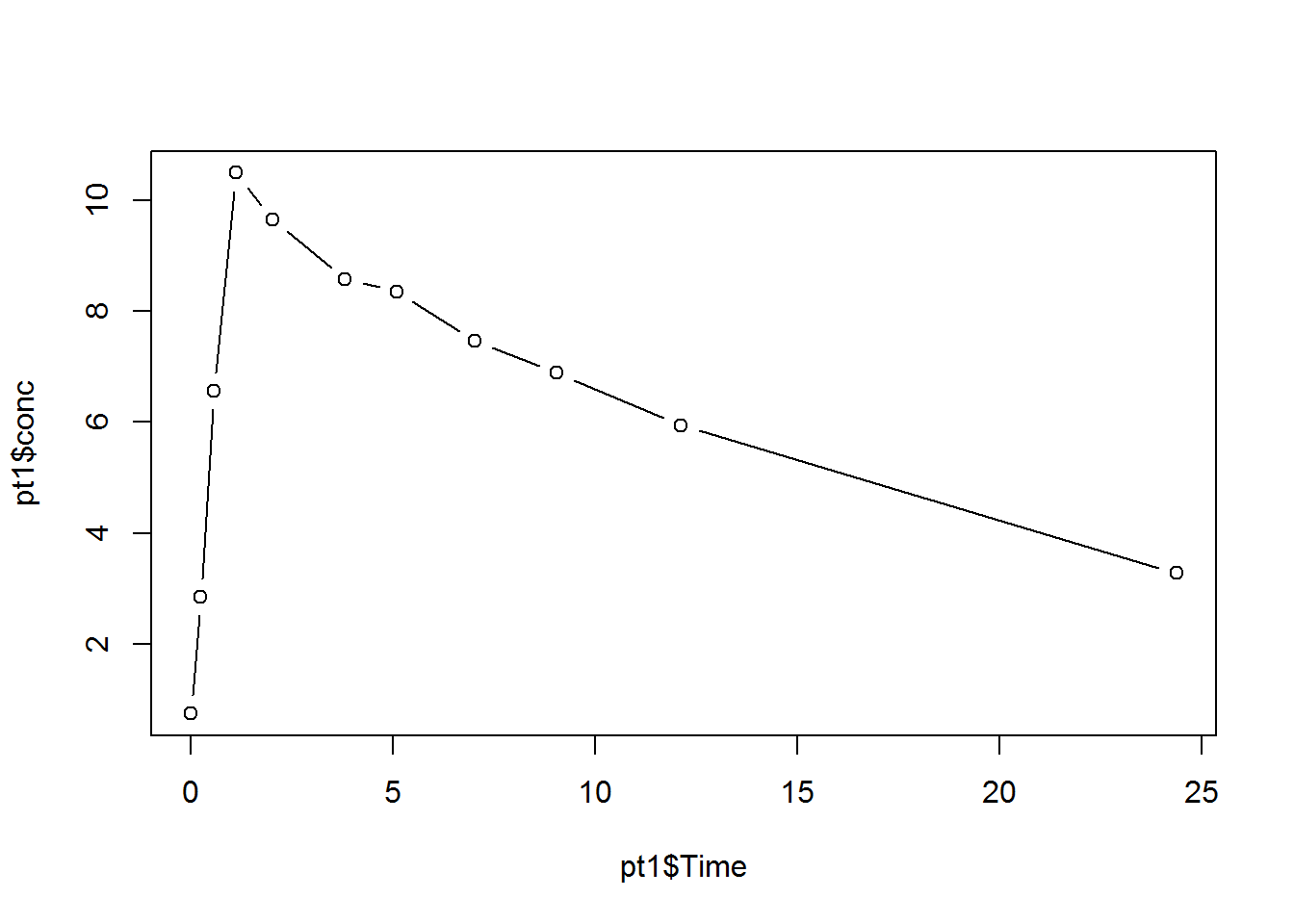``plot(pt1\$Time,log(pt1\$conc),type="b")``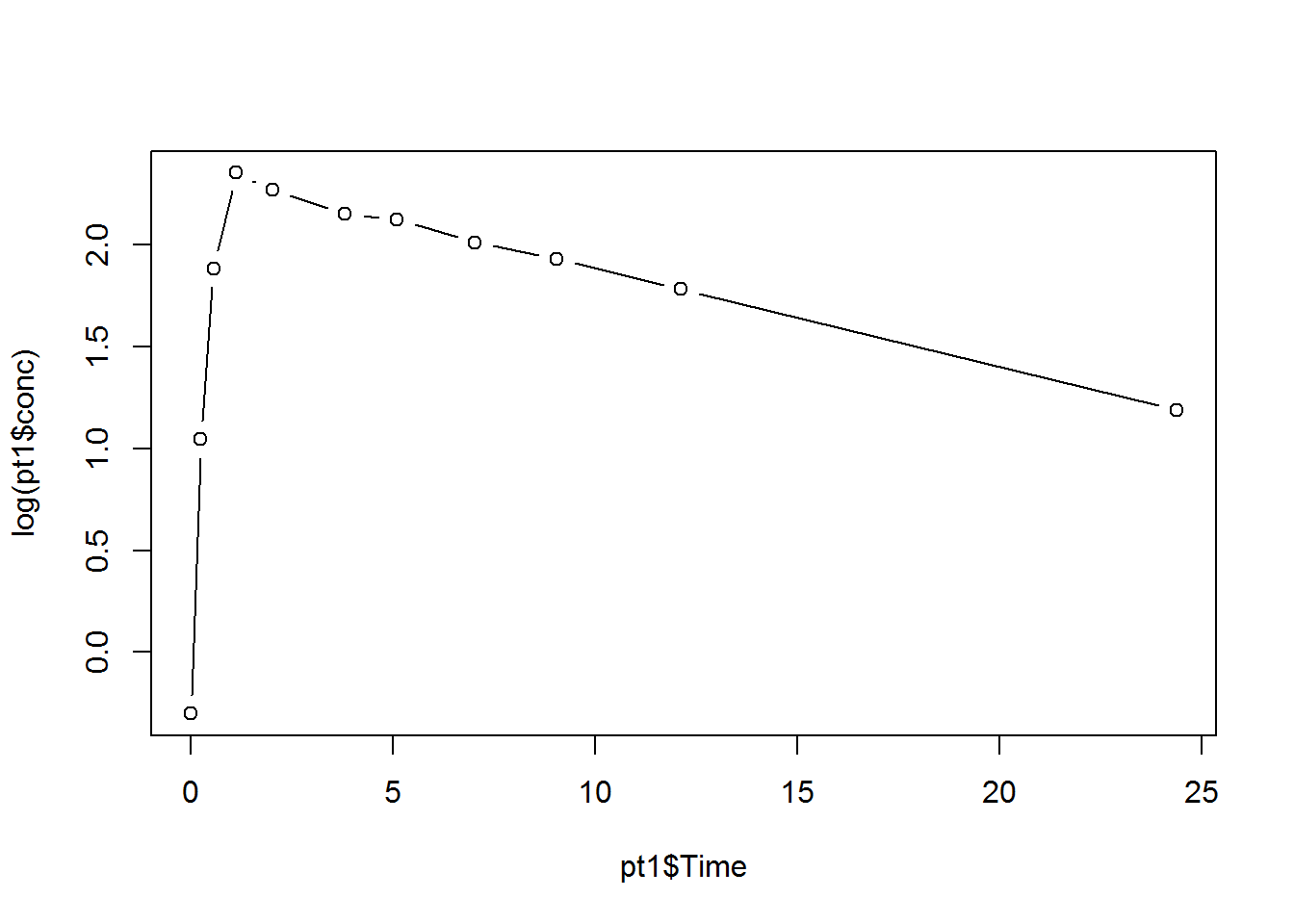This is a common pattern in many pharmacokinetic studies, particularly for oral administration of a drug.

You can use a difference in exponential fuctions as a starting point for analyzing data of this form.

``````t <- 0:350

a <- 80
b <- 1/60
c <- 1/10

y <- a*(exp(-b*t)-exp(-c*t))
plot(t,y,type="l",ylim=c(0,80),xlab=" ",ylab=" ")
title(expression(y==a*(e^{-bt}-e^{-ct})))
title(sub=paste(letters[1:3],"=",round(c(a,b,c),4),sep="",collapse=", "))``````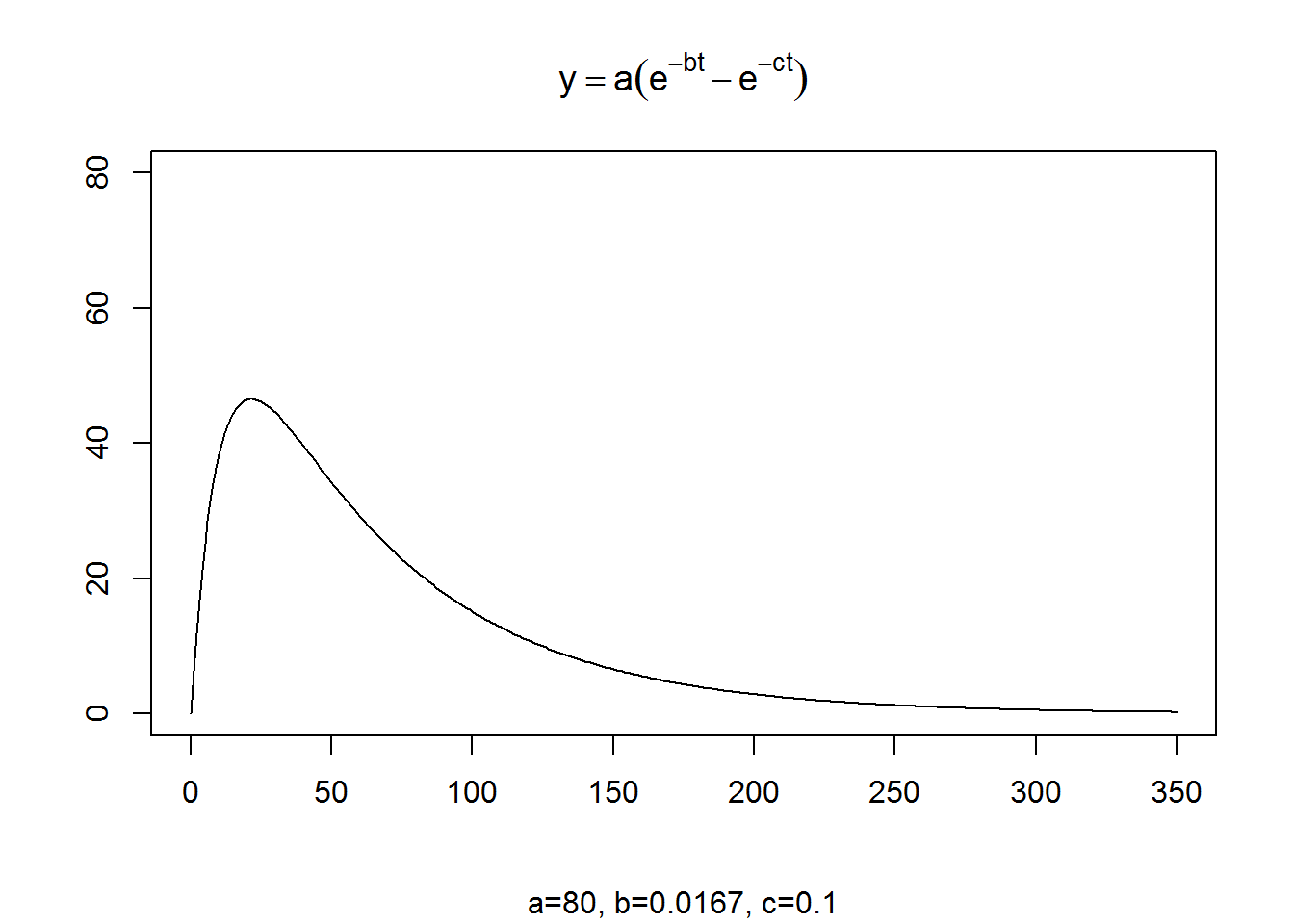You can derive this equation using differential equations. The rate at which the drug is absorbed from the gut into the bloodstream is b and the rate at which the dug is eliminated from the bloodstream by the liver and/or kidneys is c. The constant a represents the dose of the drug.

Whenever you first tackle a new nonlinear function it helps to examine how it behaves at various extremes and how it behaves as various parameters in the function change.

First note that when t=0, y=0 because \(e^{-bt}\) and \(e^{-ct}\) are both equal to 1 when t=0. Also notice that when t approaches infinity, y approaches zero again, as long as b and c are positive constants.

Also, notice that if b=c, the function equals zero everywhere. So let’s avoid the setting where b=c. It also should be apparent that b has to be smaller than c. If it is not, then the function goes negative.

This makes sense, in a way, becuase if the rate at which a drug is eliminated from the bloodstream is faster than the rate at which it is absorbed, it’s like the Monopoly game where you go straight to jail without passing go and without collecting \$200.

Likewise, a has to be a positive number for the function to represent anything meaningful.

# Examining the first derivative

Often for nonlinear functions like this, it helps to examine the first derivative, which will tell you when the function is increasing, when it is decreasing and when it reaches a minimum or maximum. You have to have at least a vague recollection of Calculus for this to make sense. If you are allergic to higher math, skip to the section “Splitting the function into two parts”.

The first derivative is \(a(-be^{-bt}+ce^{-ct})\).

The derivative equals zero at \(t=(log(c)-log(b))/(c-b)\). It is not too hard to show that the function reaches a maximum rather than a minimum here.

``````plot(t,y,type="l",ylim=c(0,80),xlab=" ",ylab=" ")
title("Location of maximum")
tmax <- (log(c)-log(b))/(c-b)
print(tmax)``````
``##  21.50111``
``````arrows(tmax,65,tmax,max(y)+5,length=0.1)
text(tmax,75,expression(frac(log(c)-log(b),c-b)))
title(sub=paste(letters[1:3],"=",round(c(a,b,c),4),sep="",collapse=", "))``````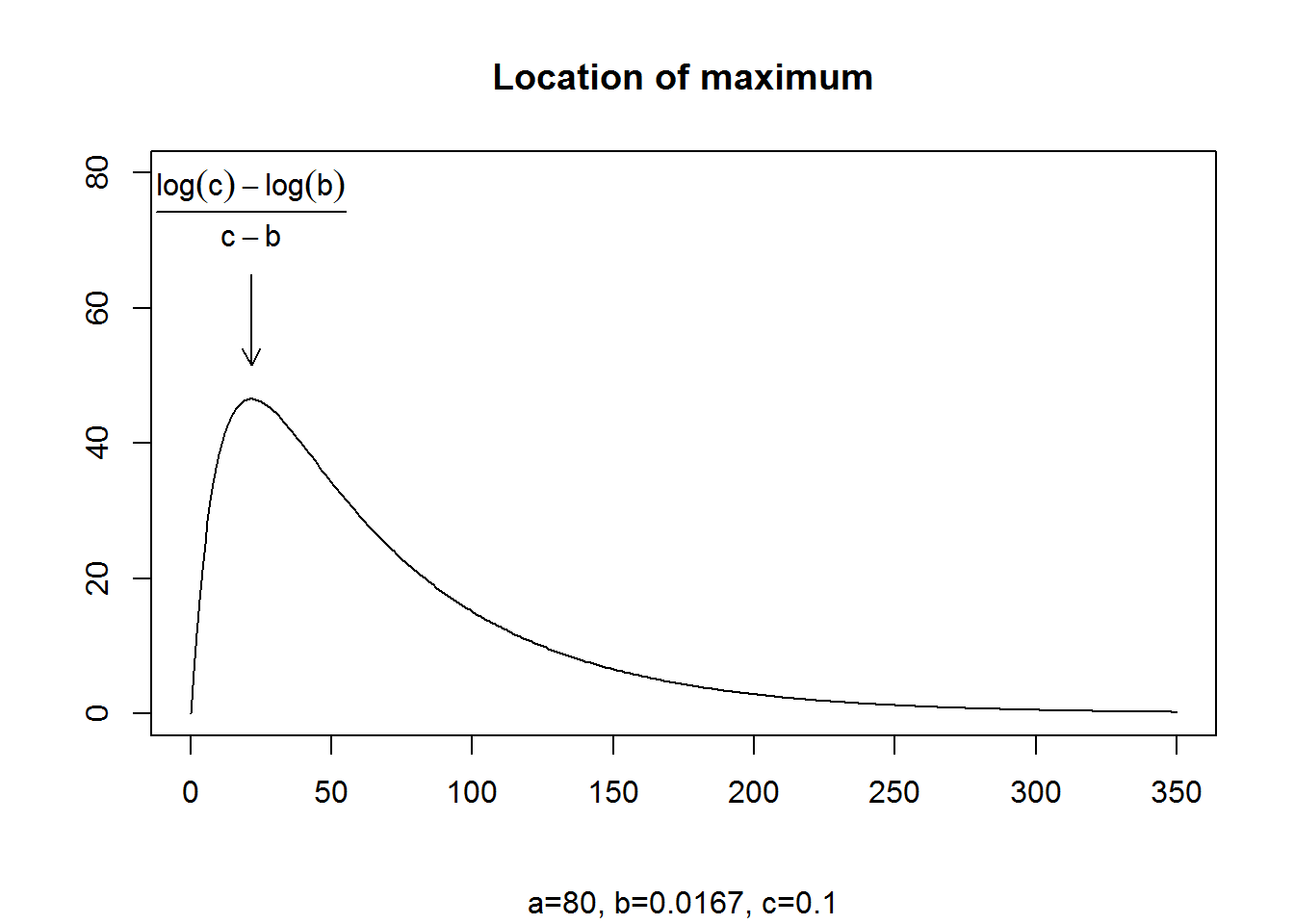The second deriviative is \(a(b^2e^{-bt}-c^2e^{-ct})\). This helps you identify when the function is convex versus concave.

The second derivative is equal to zero at \(t=2(log(c)-log(b))/(c-b)\). This is the inflection point, the point at which the function makes a transition from concave to convex.

``````plot(t,y,type="l",ylim=c(0,80),xlab=" ",ylab=" ")
title("Location of inflection point")
infl.pt <- 2*(log(c)-log(b))/(c-b)
print(infl.pt)``````
``##  43.00223``
``````y.infl <- a*(exp(-b*infl.pt)-exp(-c*infl.pt))
arrows(infl.pt,65,infl.pt,y.infl+5,length=0.1)
text(infl.pt,75,expression(2*frac(log(c)-log(b),c-b)))
title(sub=paste(letters[1:3],"=",round(c(a,b,c),4),sep="",collapse=", "))``````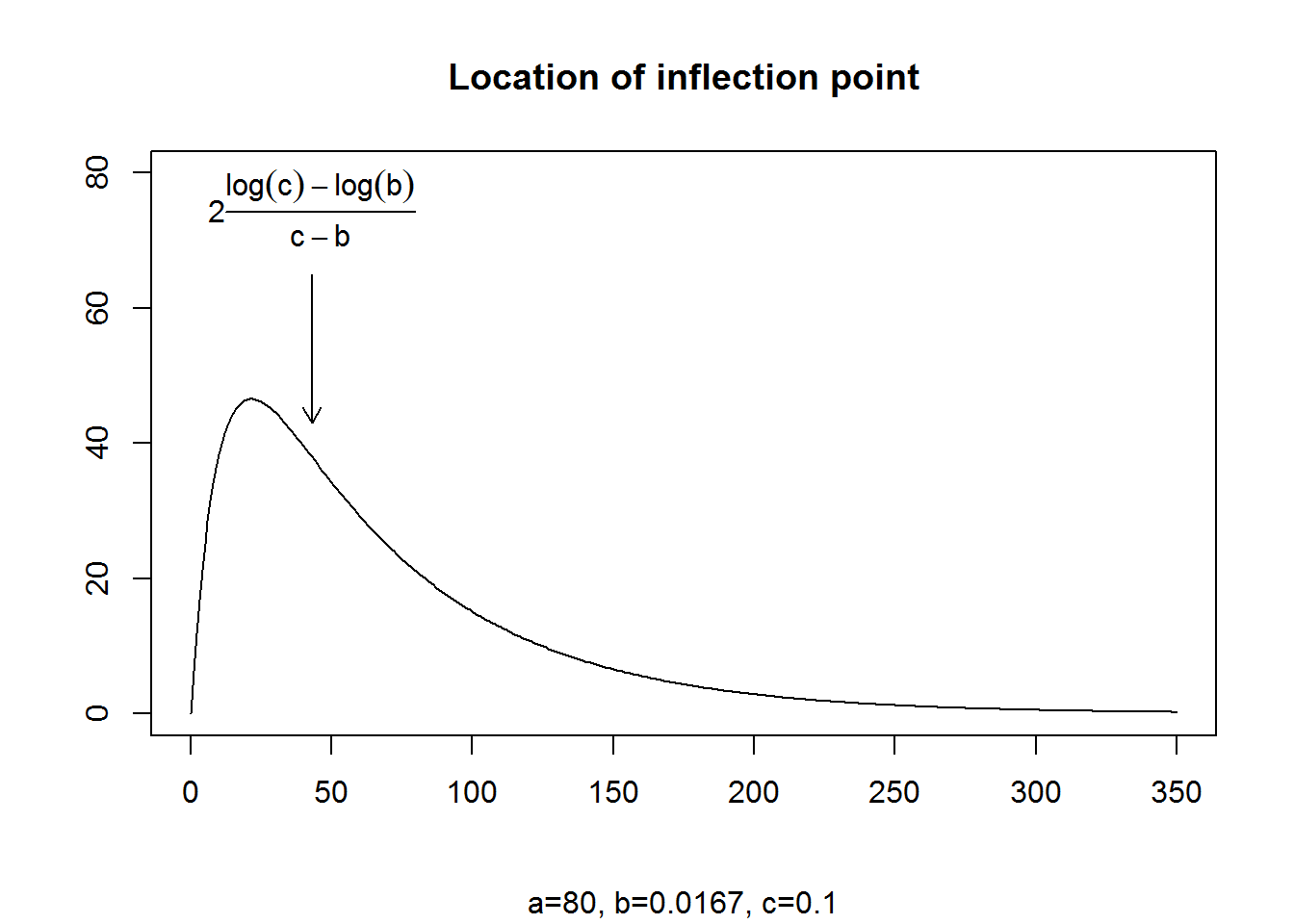## Splitting the function into two parts

It helps to split this function implicitly into two pieces. You can look at the rising portion of the function by setting the elimination rate (b) to zero, and seeing how the function changes when the absorption rate (c) changes.

``````a <- 80
b <- 1/60
c <- 1/10

y <- a*(exp(-b*t)-exp(-c*t))
plot(t,y,type="l",ylim=c(0,80),xlab=" ",ylab=" ",lty="dashed")
title(expression(y==a*(e^{-bt}-e^{-ct})))

b <- 0
y <- a*(exp(-b*t)-exp(-c*t))
lines(t,y)
title(sub=paste(letters[1:3],"=",round(c(a,b,c),4),sep="",collapse=", "))``````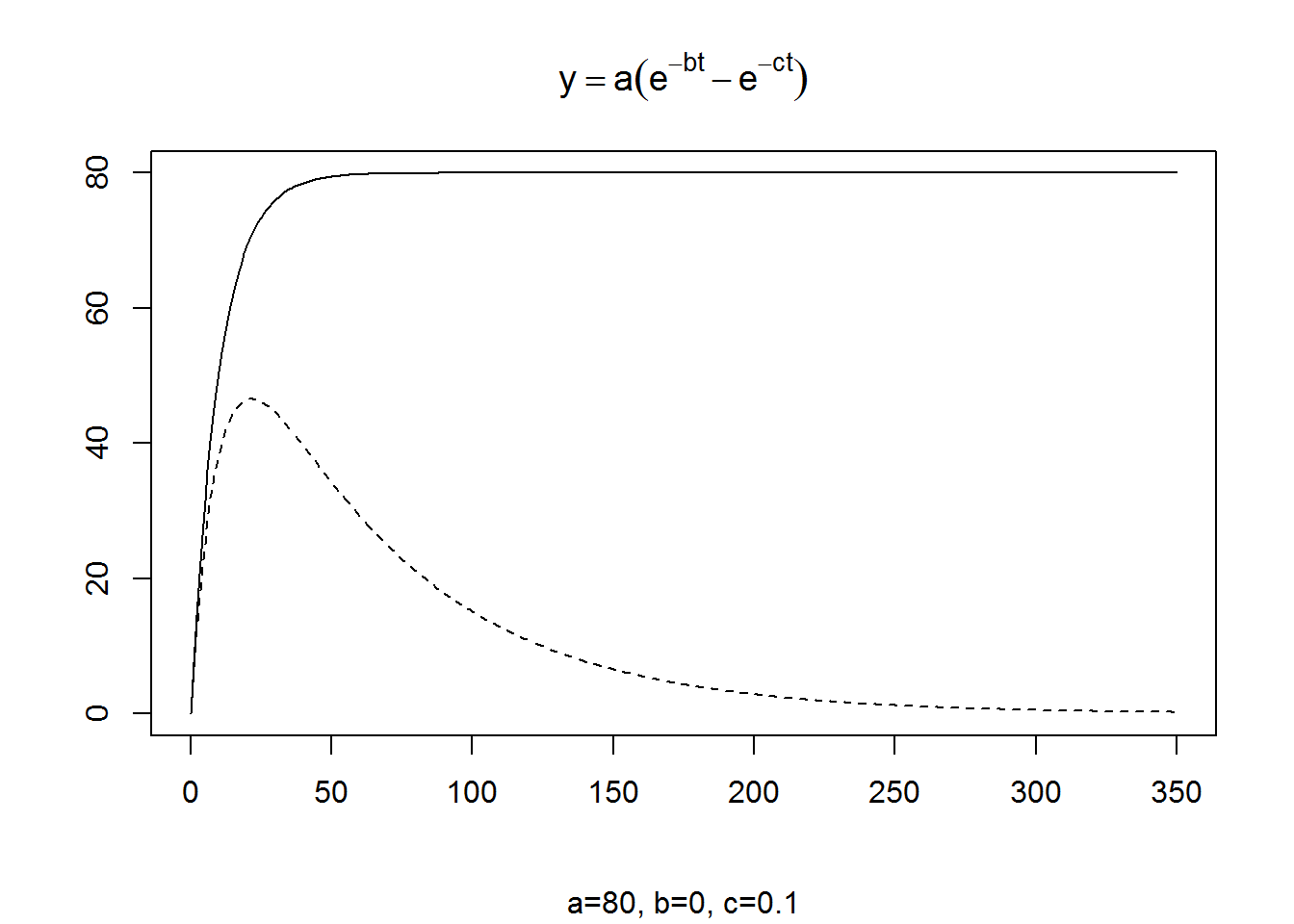The function reaches a maximum value of a (80 in our example) and the constant c controls how rapidly you rise to this maximum.

The original function with b=1/60 and c=1/10 (dashed line) does not rise to the full maximum of 80 because the liver and kidneys start chewing away on the drug the moment it first hits the bloodstream.

A little bit of algebra would show you that the simple function gets halfway to the maximum at approximately 0.7/c and 95% of the way to the maximum at 3/c.

``````a <- 80
b <- 0
c <- 1/10

y <- a*(exp(-b*t)-exp(-c*t))
plot(t,y,type="l",ylim=c(0,80),xlab=" ",ylab=" ")
title(expression(y==a*(e^{-bt}-e^{-ct})))

halfway <- 0.7/c
arrows(300,0.5*a,halfway,0.5*a,length=0.1)
pct95 <- 3/c
arrows(300,0.95*a,pct95,0.95*a,length=0.1)
title(sub=paste(letters[1:3],"=",round(c(a,b,c),4),sep="",collapse=", "))``````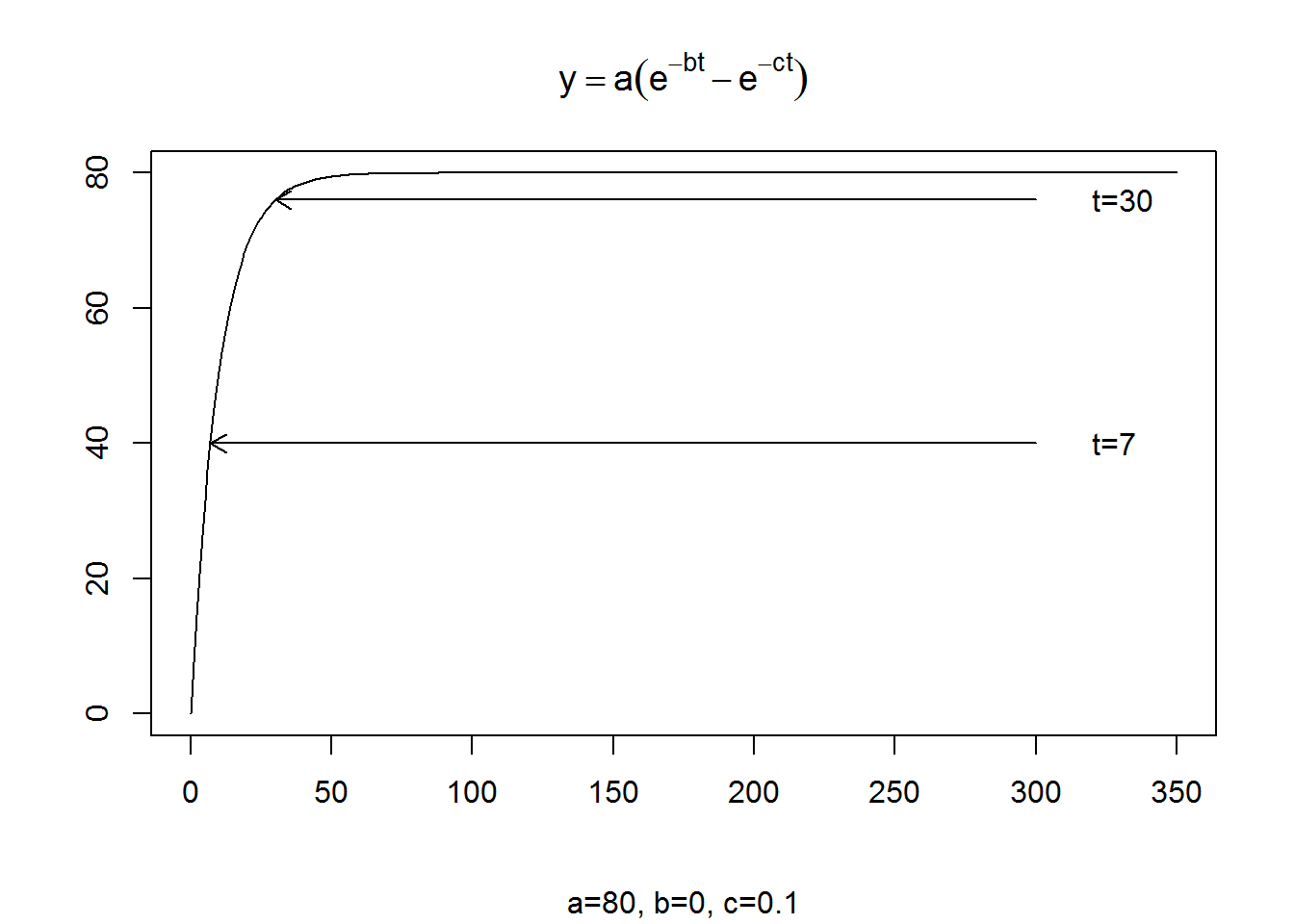The halfway point is 0.7 divided by 1/10 or 7. The curve reaches 95% of the maximum at 3 divided by 1/10 or 30.

``````a <- 80
b <- 0
c <- 1/10

y <- a*(exp(-b*t)-exp(-c*t))
plot(t,y,type="l",ylim=c(0,80),lty="dashed",xlab=" ",ylab=" ")
title(expression(y==a*(e^{-bt}-e^{-ct})))

c <- 1/30
y <- a*(exp(-b*t)-exp(-c*t))
lines(t,y)
halfway <- 0.7/c
arrows(300,0.5*a,halfway,0.5*a,length=0.1)
pct95 <- 3/c
arrows(300,0.95*a,pct95,0.95*a,length=0.1)
title(sub=paste(letters[1:3],"=",round(c(a,b,c),4),sep="",collapse=", "))``````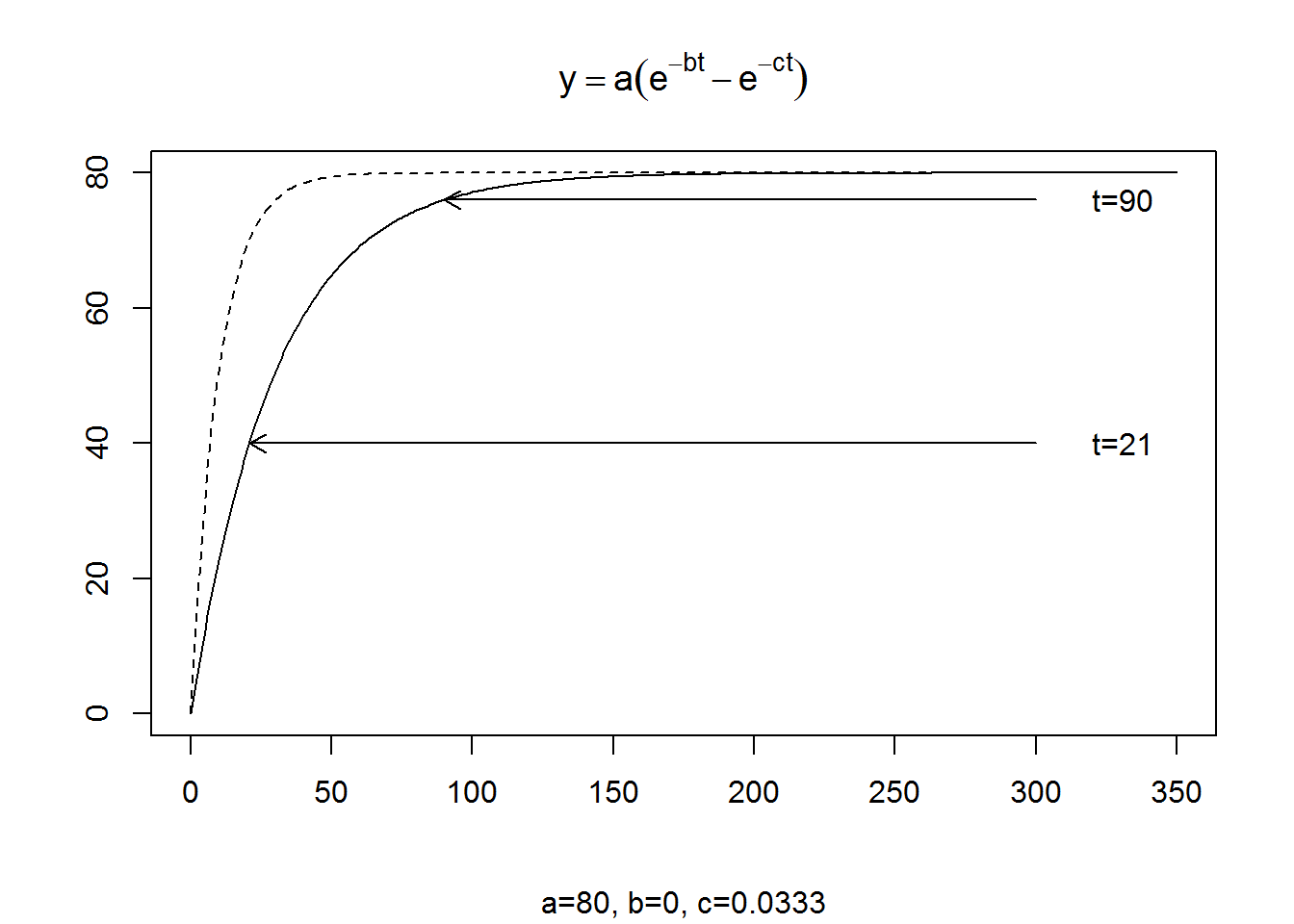For a smaller value of c, the rise occurs more slowly, getting halfway to the maximum at t=21 and 95% of the way to the maximum at t=90.

``````a <- 80
b <- 0
c <- 1/10

y <- a*(exp(-b*t)-exp(-c*t))
plot(t,y,type="l",ylim=c(0,80),lty="dashed",xlab=" ",ylab=" ")
title(expression(y==a*(e^{-bt}-e^{-ct})))

c <- 1/30
y <- a*(exp(-b*t)-exp(-c*t))
lines(t,y,lty="dashed")

c <- 1/90
y <- a*(exp(-b*t)-exp(-c*t))
lines(t,y)
halfway <- 0.7/c
arrows(300,0.5*a,halfway,0.5*a,length=0.1)
pct95 <- 3/c
arrows(300,0.95*a,pct95,0.95*a,length=0.1)
title(sub=paste(letters[1:3],"=",round(c(a,b,c),4),sep="",collapse=", "))``````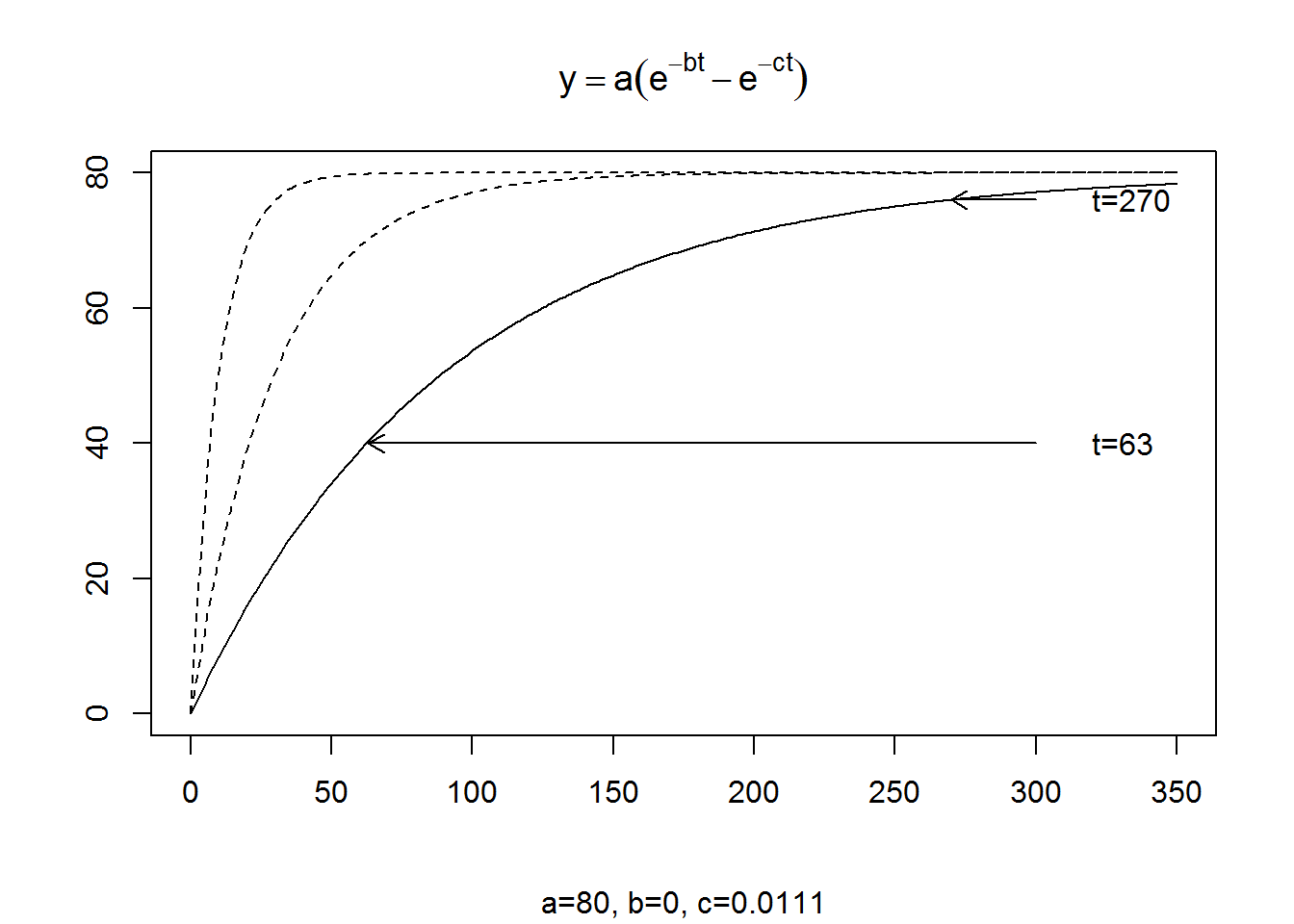For an ever smaller value of c (1/90), the halfway point (t=63) and the 95% point (t=270) take even longer.

Now let’s think about the other half of the equation, the elimination half. Let’s assume the absorption takes place more or less instantaneously, by setting c equal to infinity. With an infinite c, the term \(e^{-ct}\) becomes zero, and the equation simplifies to \(ae^{-bt}\). In R, you can’t put in a value of infinity, but any reasonably large number will be a good enough approximation.A value of c=100, for example, would be equivalent to having 95% of the absorption occur before t=0.03.

``````a <- 80
b <- 1/60
c <- 1/10

y <- a*(exp(-b*t)-exp(-c*t))
plot(t,y,type="l",ylim=c(0,80),xlab=" ",ylab=" ",lty="dashed")
title(expression(y==a*(e^{-bt}-e^{-ct})))

c <- 100
y <- a*(exp(-b*t)-exp(-c*t))
y <- a
lines(t,y)
title(sub=paste(letters[1:3],"=",round(c(a,b,c),4),sep="",collapse=", "))``````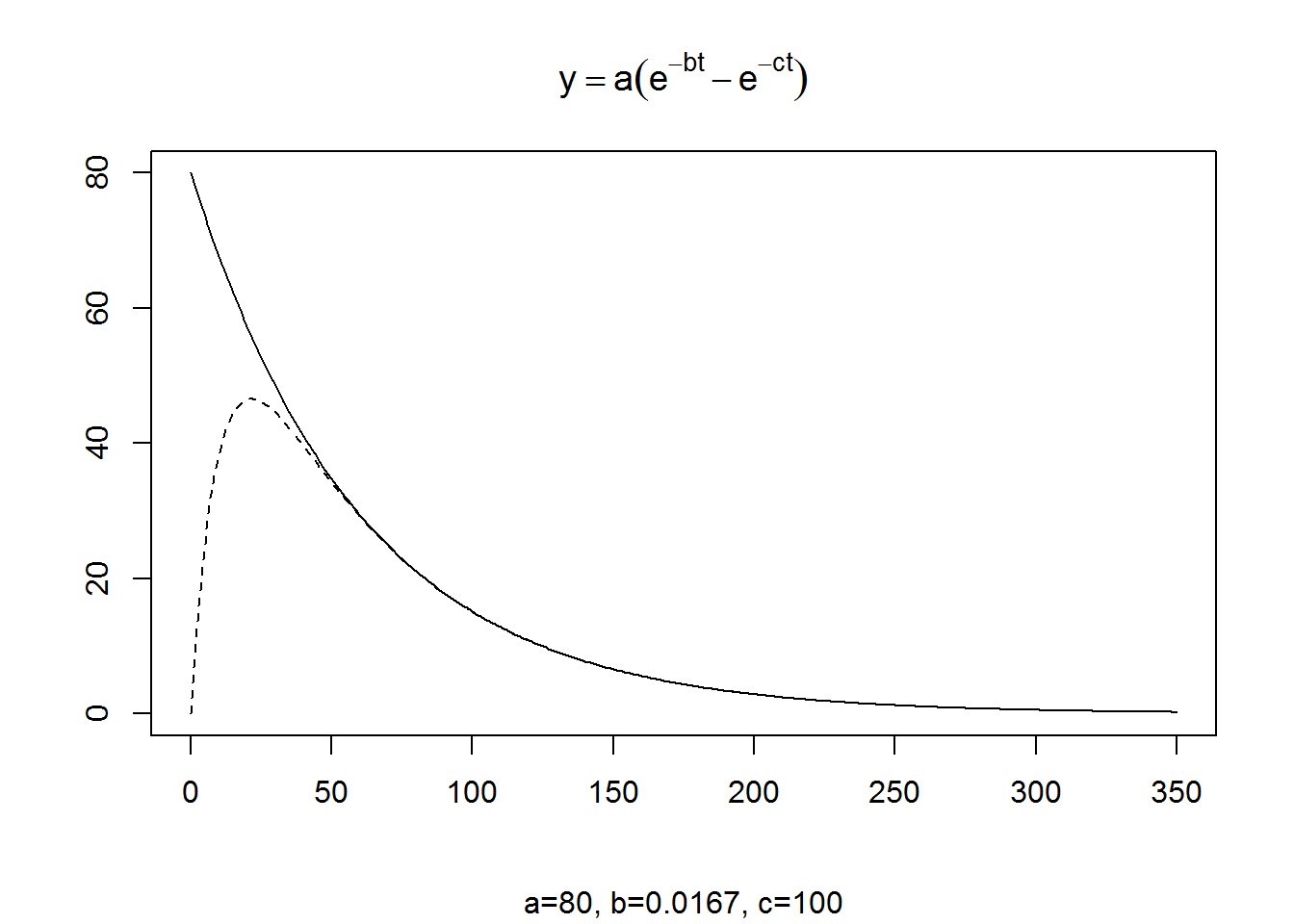The point at which half of the drug is eliminated is approximately 0.7/b and the point at which 95% of the drug is elimiated is approximately 3/b.

``````a <- 80
b <- 1/60
c <- 100
y <- a*(exp(-b*t)-exp(-c*t))
y <- a
plot(t,y,type="l",ylim=c(0,80),xlab=" ",ylab=" ")
title(expression(y==a*(e^{-bt}-e^{-ct})))
halfway <- 0.7/b
arrows(300,0.5*a,halfway,0.5*a,length=0.1)
pct95 <- 3/b
arrows(300,0.05*a,pct95,0.05*a,length=0.1)
title(sub=paste(letters[1:3],"=",round(c(a,b,c),4),sep="",collapse=", "))``````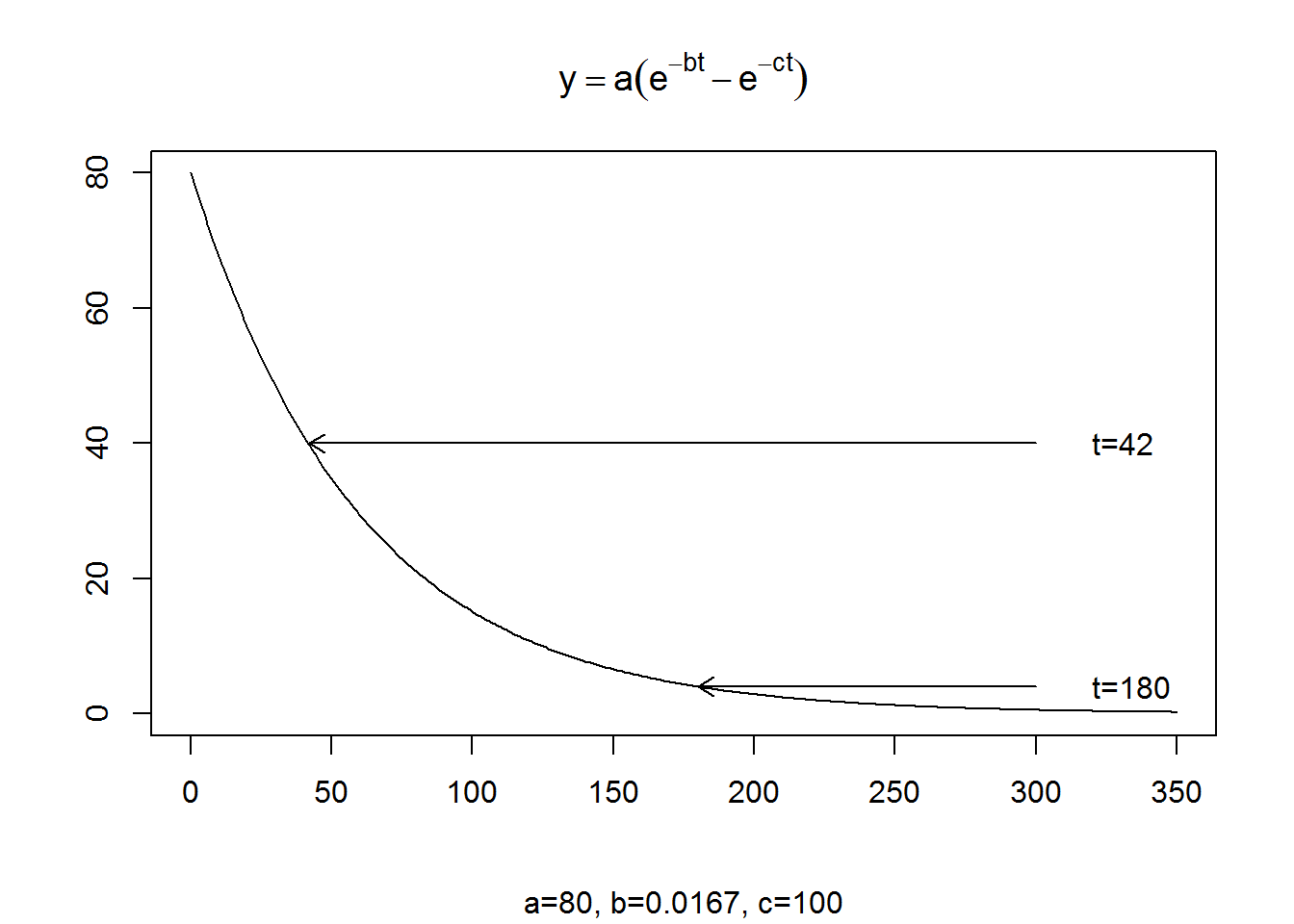In this example, these values are 42 and 180. For smaller values of b, it takes even longer to eliminate the drug from your body. For larger values of b, it takes less time to eliminate the drug from your body.

``````a <- 80
b <- 1/60
c <- 100
y <- a*(exp(-b*t)-exp(-c*t))
y <- a
plot(t,y,type="l",ylim=c(0,80),xlab=" ",ylab=" ",lty="dashed")
title(expression(y==a*(e^{-bt}-e^{-ct})))

a <- 80
b <- 1/180
c <- 100
y <- a*(exp(-b*t)-exp(-c*t))
y <- a
lines(t,y)

halfway <- 0.7/b
arrows(50,0.5*a,halfway,0.5*a,length=0.1)
pct95 <- 3/b
arrows(50,0.05*a,350,0.05*a,length=0.1)
title(sub=paste(letters[1:3],"=",round(c(a,b,c),4),sep="",collapse=", "))``````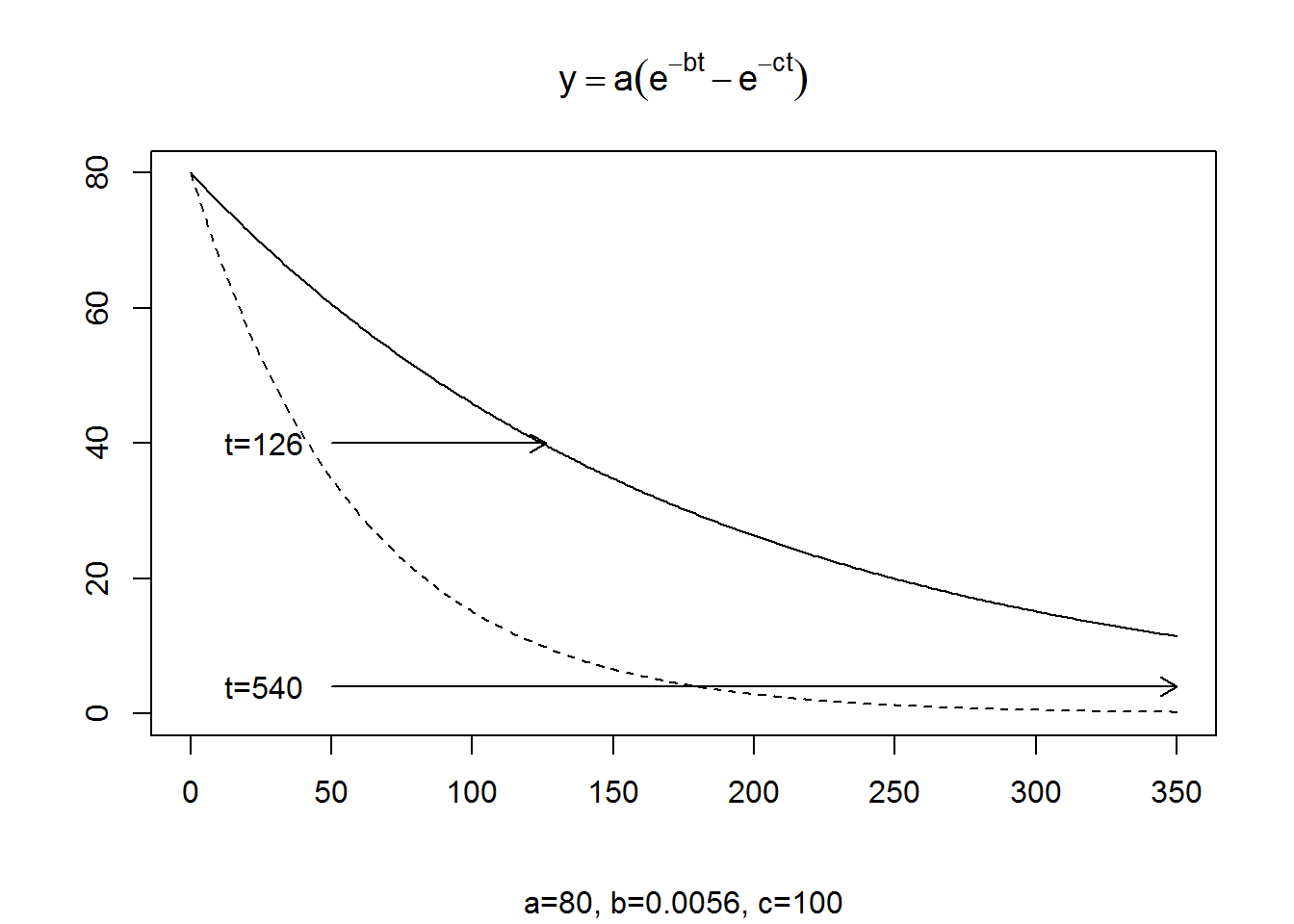When b decreases from 1/60 to 1/180, hlaf thedrug is eliminated at t=126 and 95% of the drug is eliminated at t=540 (which is off the scale of this graph).

## Interpretation of a

a represents the maximum theoretical concentration of the drug. Note that the concentration of the drug is not the same as the dose of the drug. When you are running a pharmacokinetic experiment, you don’t take every last ounce of blood out to see how much total drug there is (was) in your body. You take a small amount and measure the concentration of the drug. So while the dose might be measured in micrograms, the concentration is measured in micrograms per liter or some similar unit.

The total dose should be equal to the volume of blood times the concentration. The typical adult has about 5 liters of blood. To be a bit more precise, you have consider the volume of both the blood and the rapidly perfused tissues. If D is the dose, then D=V*a where V is called the volume of distribution.

The volume of distribution is closely related to weight, so often this number is computed as micrograms per liter per kilogram of body weight. The volume of distribution can change markedly. A drug that is highly fat soluble usually has a much larger volume of distribution because it gets distributed across many more parts of your body.

I know that I am oversimplifying things, partly to make the more readable and partly because I don’t know enough about physiology, chemistry, etc. to explain it better.

## Applying this model to the first Theophyllin patient.

Let’s look at the Theophyllin data again.

``plot(pt1\$Time,pt1\$conc,type="b")``Let’s try to find values of a, b, and c that model this relationship well.

The highest concentration is 10.5, so the theoretical maximum, a, has to be at least this large.

The drug reaches about half of the actual maximum at t=0.57. So solve the equation 0.57=0.7/c to get c=1.23.

The drug falls to about half of its maximum a bit beyond t=12.12. Solve the equation 12.12=0.7/b to get b=0.057.

Now, when b and c are close to one another, the theoretical maximum is a lot higher than the observed maximum. Here, they’re not that close to one another and we’re just trying to get a rough feel for things. So let’s start with a=12, b=0.057 and c=1.23.

Our first guess may be way off, so let’s give ourselves a bit of room on the graph by extending the y-axis to about three times the maximum observed value.

``````plot(pt1\$Time,pt1\$conc,type="b",ylim=c(0,30))
a <- 12
b <- 0.057
c <- 1.23
t <- seq(0,24,by=0.01)
y <- a*(exp(-b*t)-exp(-c*t))
lines(t,y)``````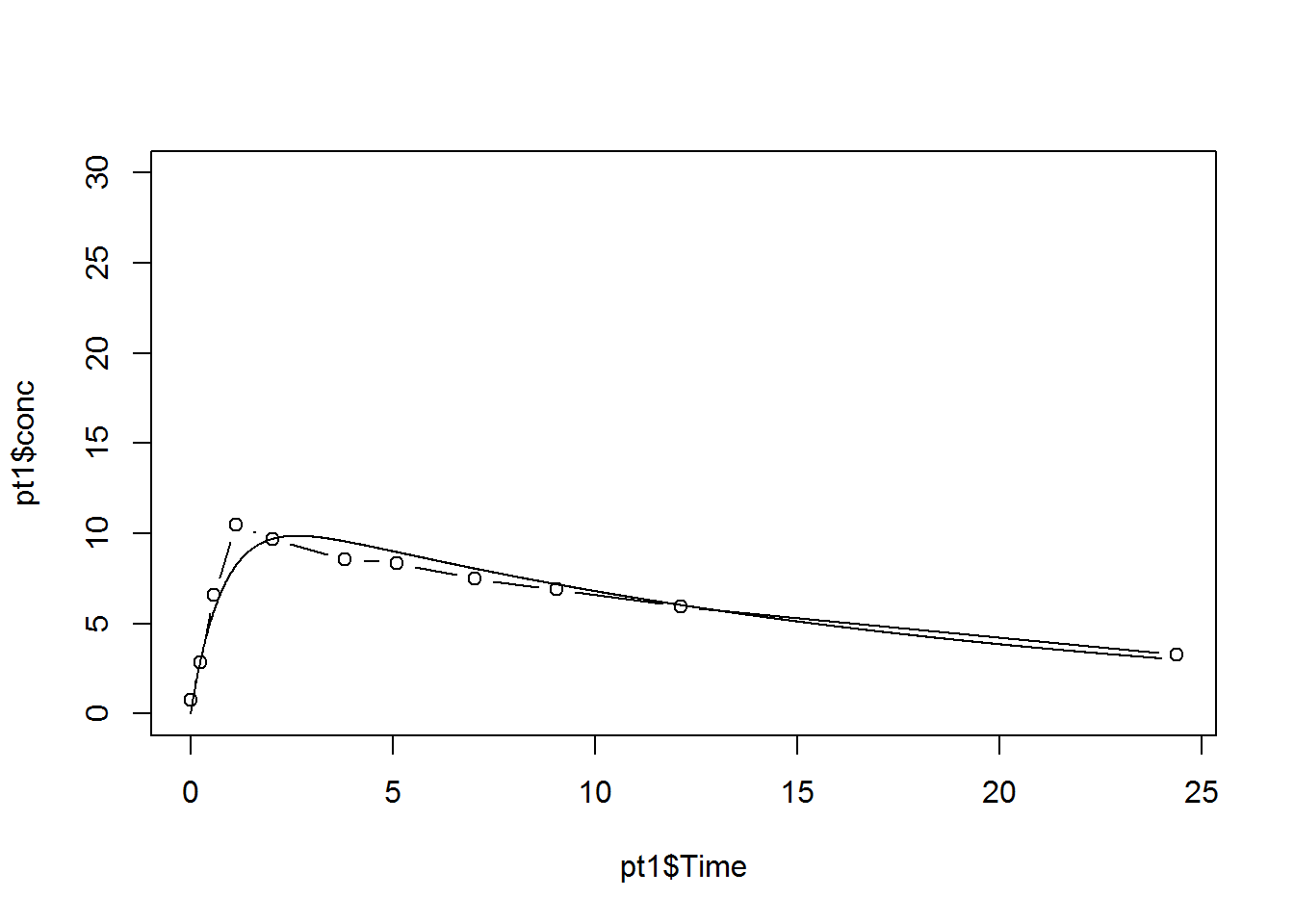``print(max(y))``
``##  9.857099``
``print(t[y==max(y)])``
``##  2.62``

This is not too bad. The absorption rate might be a bit faster.

``````plot(pt1\$Time,pt1\$conc,type="b",ylim=c(0,30))
a <- 12
b <- 0.057
c <- 2.5
y <- a*(exp(-b*t)-exp(-c*t))
lines(t,y)``````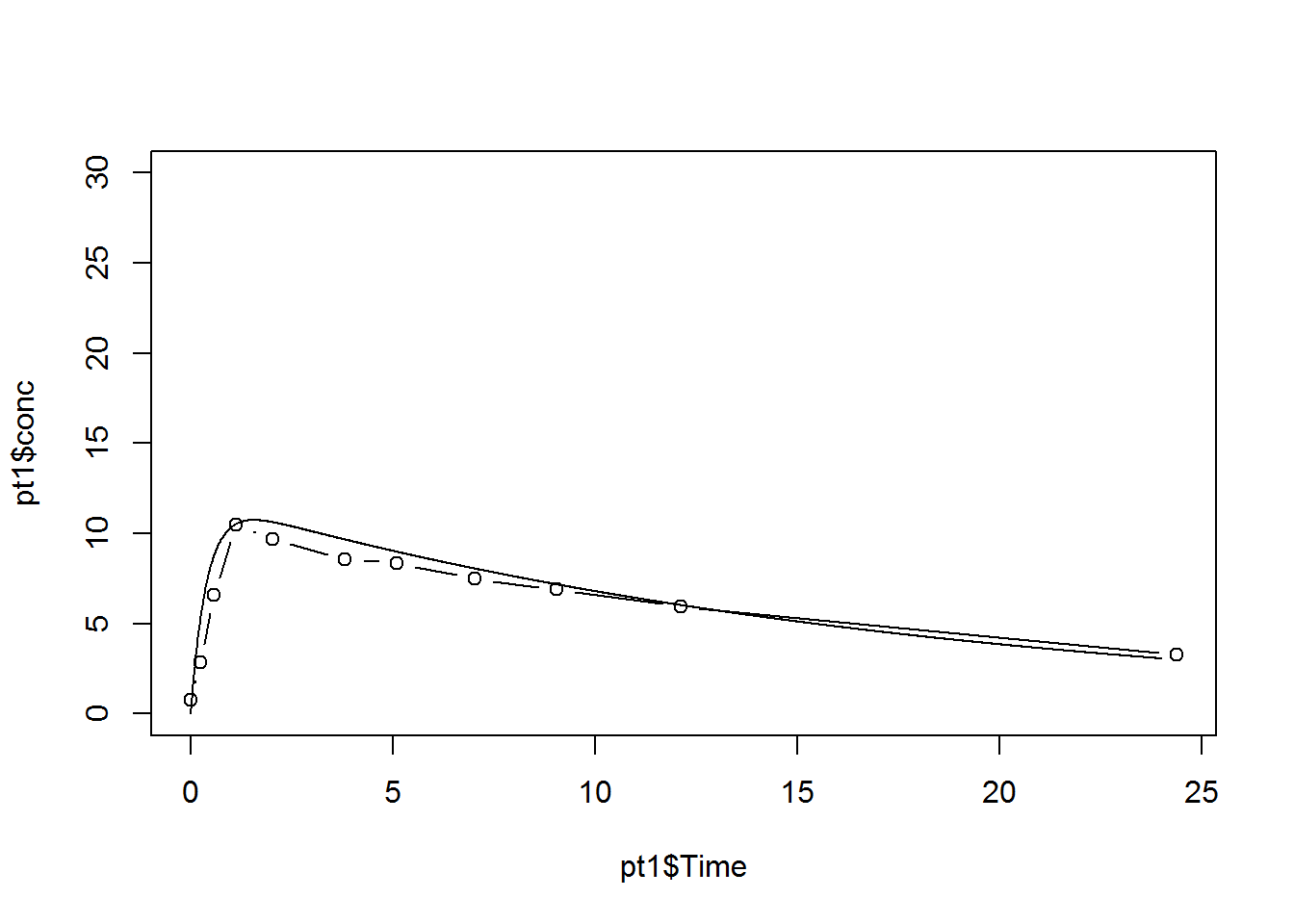``print(max(y))``
``##  10.73623``
``print(t[y==max(y)])``
``##  1.55``

Still not fast enough. Also the elimination rate looks a bit too fast.

``````plot(pt1\$Time,pt1\$conc,type="b",ylim=c(0,30))
a <- 12
b <- 0.09
c <- 3.75
y <- a*(exp(-b*t)-exp(-c*t))
lines(t,y)``````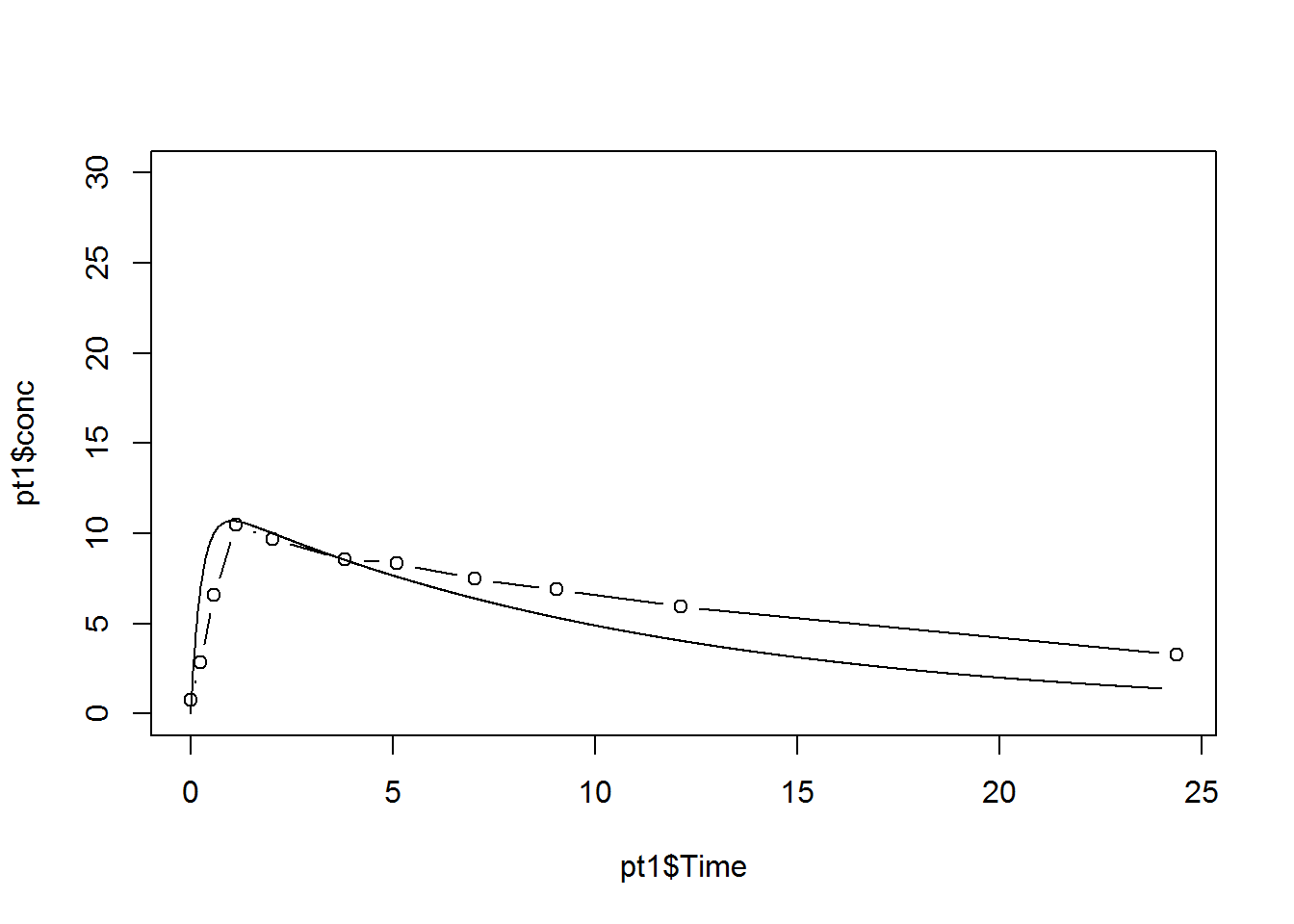``print(max(y))``
``##  10.68563``
``print(t[y==max(y)])``
``##  1.02``

Let’s slow down the elimination rate a bit more.

``````plot(pt1\$Time,pt1\$conc,type="b",ylim=c(0,30))
a <- 12
b <- 0.04
c <- 3.75
y <- a*(exp(-b*t)-exp(-c*t))
lines(t,y)``````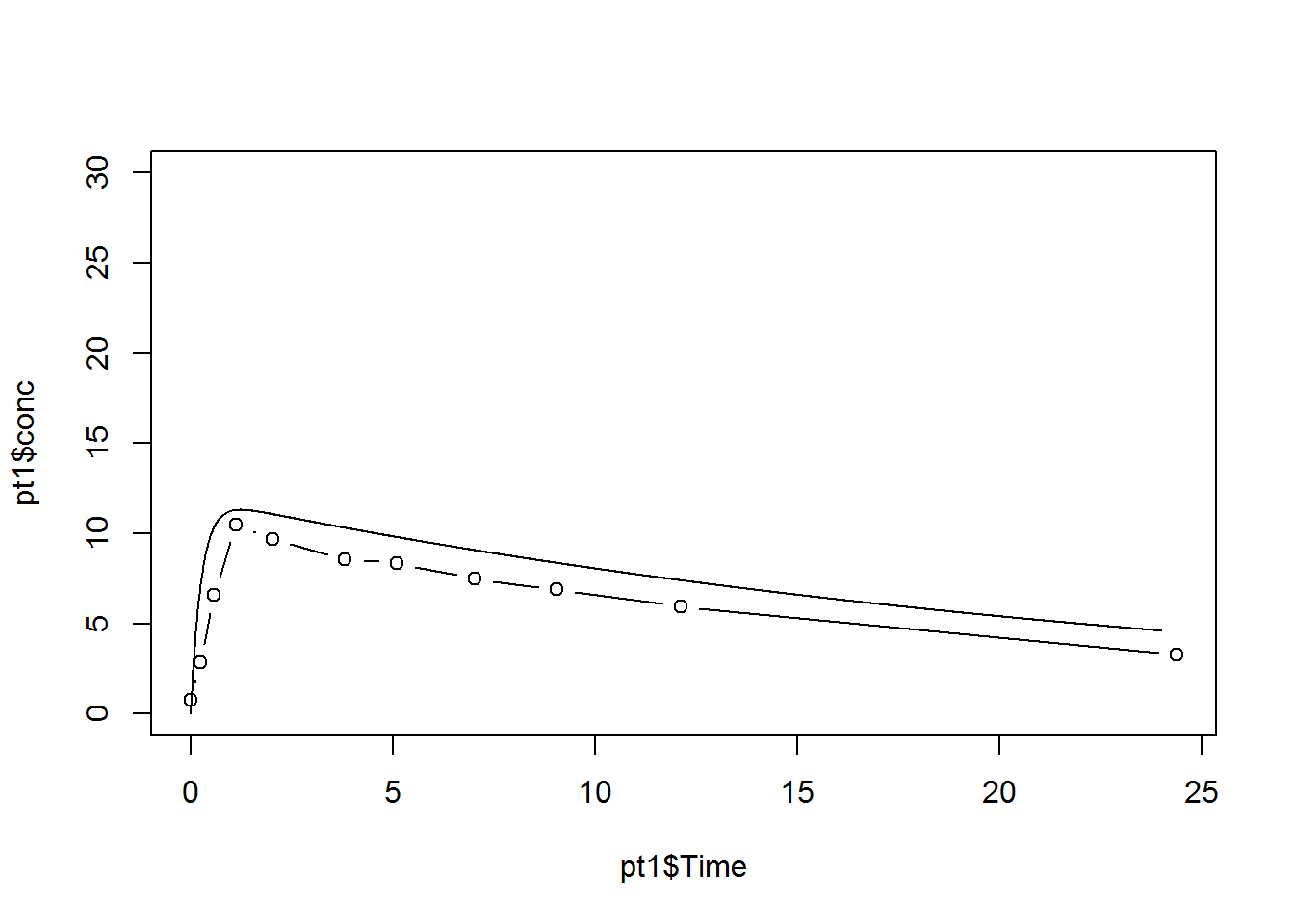``print(max(y))``
``##  11.30478``
``print(t[y==max(y)])``
``##  1.22``

Notice that we’re overshooting but the downhill phase does look to be parallel. Let’s lower the overall height by reducting a.

``````plot(pt1\$Time,pt1\$conc,type="b",ylim=c(0,30))
a <- 10
b <- 0.04
c <- 3.75
y <- a*(exp(-b*t)-exp(-c*t))
lines(t,y)``````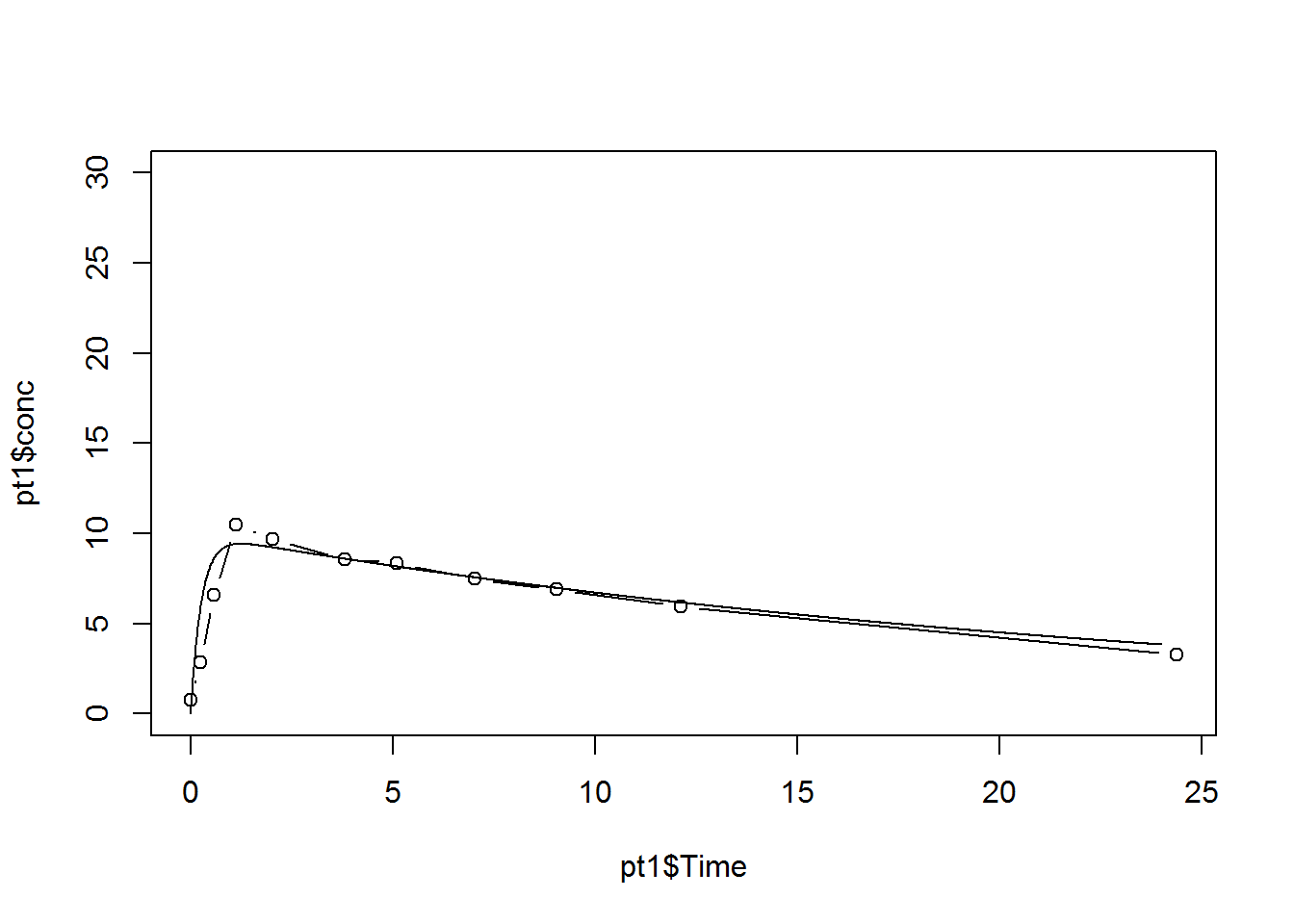``print(max(y))``
``##  9.420653``
``print(t[y==max(y)])``
``##  1.22``

This is looking very good. You might want to decreate the absorption rate a bit.

Now in practice, you should not have to do this trial and error approach. Just try it a couple of times with your data set to get comfortable with how to shift, squeeze, or stretch the curve to make it come close to the data points.

There are some better tools for estimating the absorption and elimination rates that involve looking at slopes of straight line fits to the log concentrations.

``````first.half <- coef(lm(log(conc[1:4])~Time[1:4],data=pt1))
second.half <- coef(lm(log(conc[5:11])~Time[5:11],data=pt1))
plot(pt1\$Time,log(pt1\$conc),type="p")
abline(first.half)
abline(second.half)``````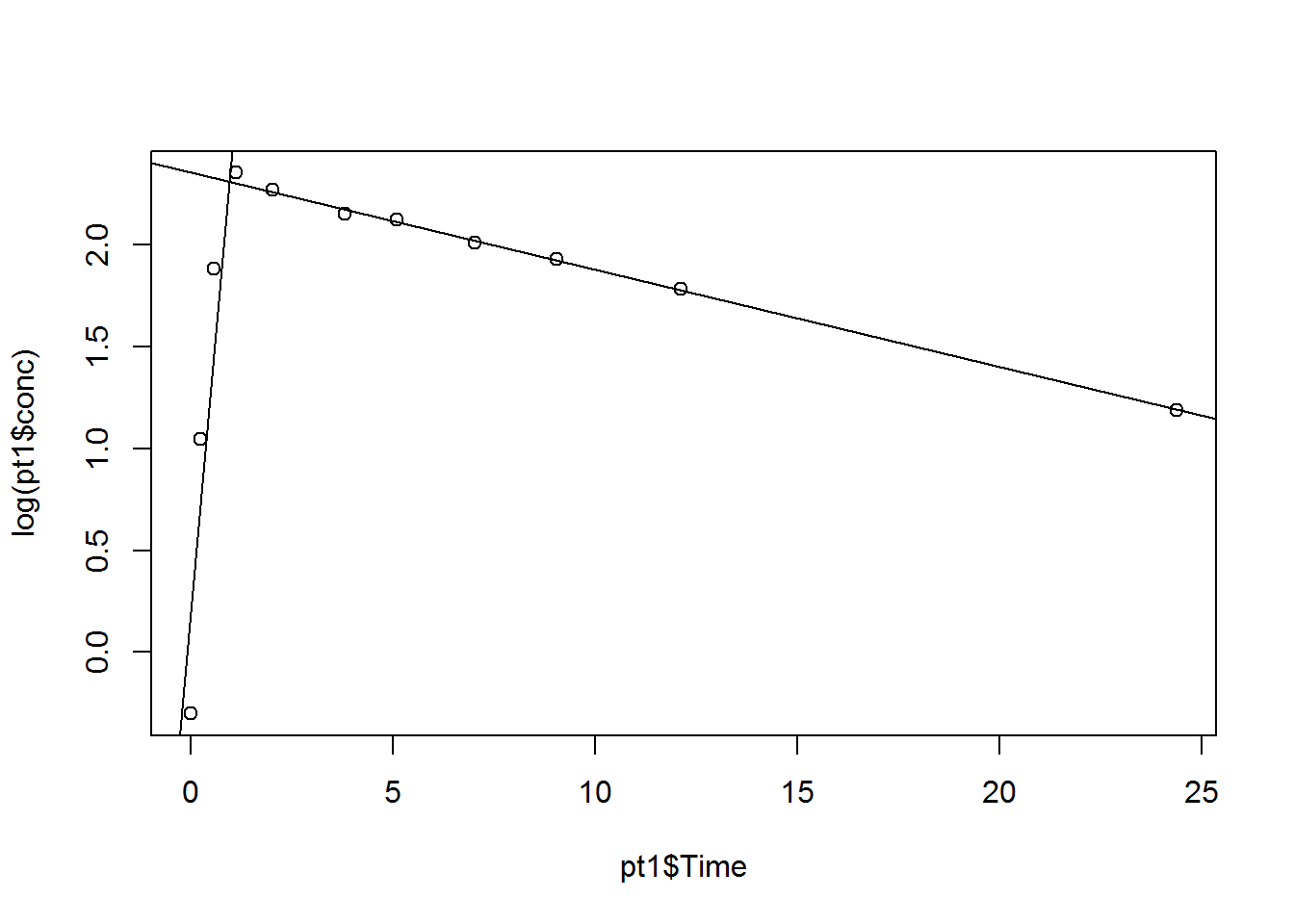``print(first.half)``
``````## (Intercept)   Time[1:4]
##   0.1689133   2.2169767``````
``print(second.half)``
``````## (Intercept)  Time[5:11]
##  2.35518701 -0.04778625``````

But better still is to use a nonlinear regression fitting algorithm.

``````start.vector <- c(a=12,b=0.057,c=1.23)
mod1 <- nls(conc~a*(exp(-b*Time)-exp(-c*Time)),data=pt1,
start=start.vector,trace=TRUE)``````
``````## 8.302106 :  12.000  0.057  1.230
## 5.140659 :  10.56850058  0.04881868  1.79284712
## 4.286761 :  11.20364358  0.05375007  1.78008835
## 4.286013 :  11.22796145  0.05395621  1.77679448
## 4.286009 :  11.22697804  0.05395134  1.77760814
## 4.286009 :  11.22742333  0.05395539  1.77735570
## 4.286009 :  11.22729609  0.05395429  1.77743119
## 4.286009 :  11.22733466  0.05395463  1.77740848``````
``print(mod1)``
``````## Nonlinear regression model
##   model: conc ~ a * (exp(-b * Time) - exp(-c * Time))
##    data: pt1
##        a        b        c
## 11.22733  0.05395  1.77741
##  residual sum-of-squares: 4.286
##
## Number of iterations to convergence: 7
## Achieved convergence tolerance: 7.903e-06``````
``summary(mod1)``
``````##
## Formula: conc ~ a * (exp(-b * Time) - exp(-c * Time))
##
## Parameters:
##   Estimate Std. Error t value Pr(>|t|)
## a 11.22734    0.76814  14.616 4.71e-07 ***
## b  0.05396    0.00922   5.852 0.000382 ***
## c  1.77741    0.30716   5.787 0.000411 ***
## ---
## Signif. codes:  0 '***' 0.001 '**' 0.01 '*' 0.05 '.' 0.1 ' ' 1
##
## Residual standard error: 0.732 on 8 degrees of freedom
##
## Number of iterations to convergence: 7
## Achieved convergence tolerance: 7.903e-06``````
``````yp <- predict(mod1,newdata=data.frame(Time=t))
plot(pt1\$Time,pt1\$conc,type="p")
lines(t,yp)``````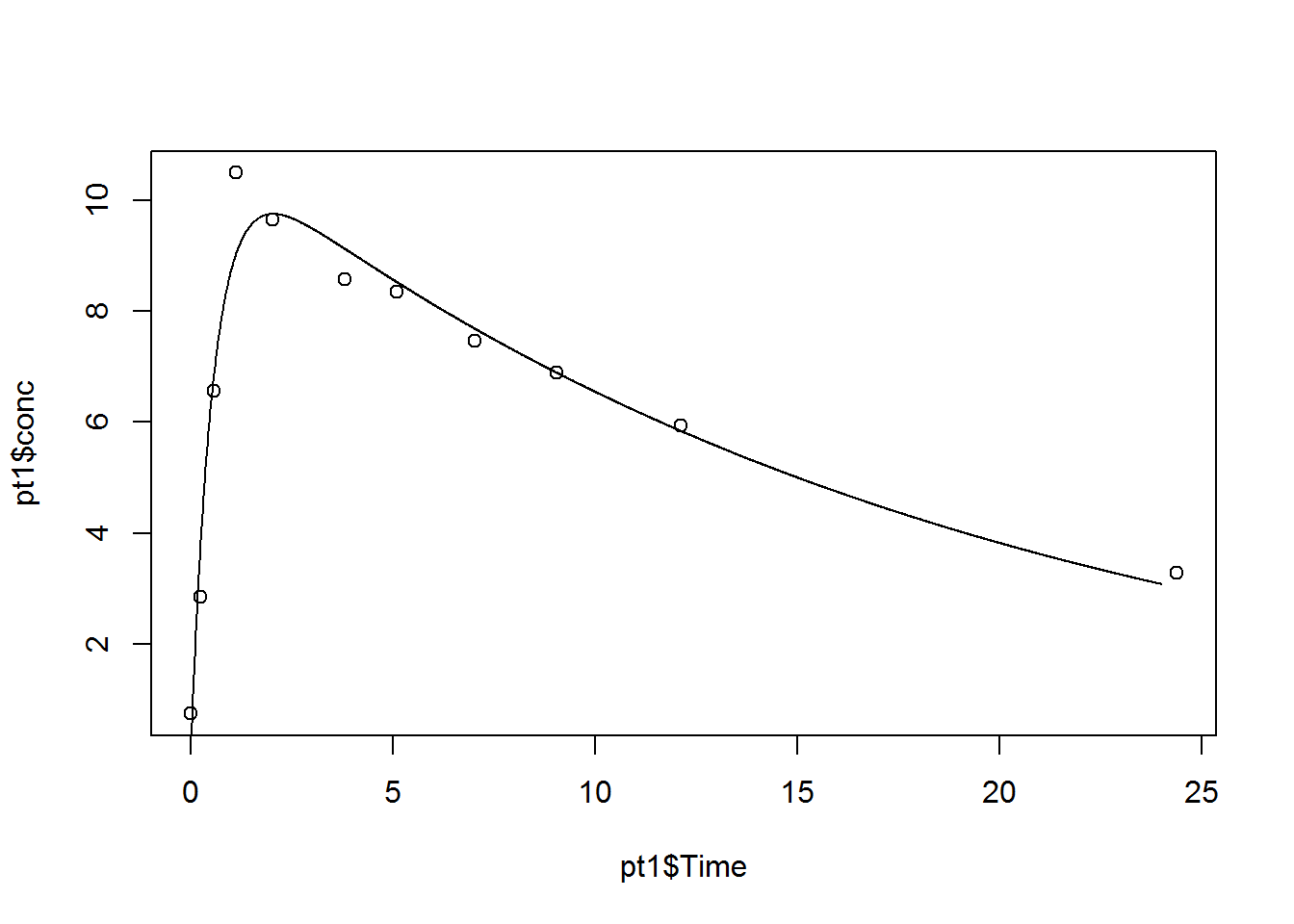The starting values are critical here. If you use bad starting values, you may not get convergence or you may converge to a bad solution.

``````start.vector <- c(a=12,b=0.57,c=1.23)
tryCatch(nls(conc~a*(exp(-b*Time)-exp(-c*Time)),data=pt1,
start=start.vector),error=function(e) e)``````
``## <simpleError in nls(conc ~ a * (exp(-b * Time) - exp(-c * Time)), data = pt1,     start = start.vector): singular gradient>``

R has some nice commonly used nonlinear functions with special features like the ability to generate reasonable starting values for a particular data set. These functions have the prefix SS for self-starting. Type ?selfStart for more details.

The particular nonlinear function that you want here is SSfol.

``````mod2 <- nls(conc~SSfol(Dose,Time,lke,lka,lcl),data=pt1,
trace=TRUE)``````
``````## 4.388837 :  -2.994845  0.609169 -3.971003
## 4.287881 :  -2.9169766  0.5669543 -3.9141570
## 4.286156 :  -2.9209078  0.5776641 -3.9165716
## 4.286022 :  -2.9192534  0.5744082 -3.9156613
## 4.28601 :  -2.9197233  0.5753851 -3.9159158
## 4.286009 :  -2.9195804  0.5750916 -3.9158382
## 4.286009 :  -2.9196232  0.5751797 -3.9158615
## 4.286009 :  -2.9196103  0.5751532 -3.9158545
## 4.286009 :  -2.9196142  0.5751612 -3.9158566``````
``print(mod2)``
``````## Nonlinear regression model
##   model: conc ~ SSfol(Dose, Time, lke, lka, lcl)
##    data: pt1
##     lke     lka     lcl
## -2.9196  0.5752 -3.9159
##  residual sum-of-squares: 4.286
##
## Number of iterations to convergence: 8
## Achieved convergence tolerance: 4.907e-06``````
``summary(mod2)``
``````##
## Formula: conc ~ SSfol(Dose, Time, lke, lka, lcl)
##
## Parameters:
##     Estimate Std. Error t value Pr(>|t|)
## lke  -2.9196     0.1709 -17.085 1.40e-07 ***
## lka   0.5752     0.1728   3.328   0.0104 *
## lcl  -3.9159     0.1273 -30.768 1.35e-09 ***
## ---
## Signif. codes:  0 '***' 0.001 '**' 0.01 '*' 0.05 '.' 0.1 ' ' 1
##
## Residual standard error: 0.732 on 8 degrees of freedom
##
## Number of iterations to convergence: 8
## Achieved convergence tolerance: 4.907e-06``````
``````yp <- predict(mod2,newdata=data.frame(Dose=rep(pt1\$Dose,length(t)),Time=t))
plot(pt1\$Time,pt1\$conc,type="p")
lines(t,yp)``````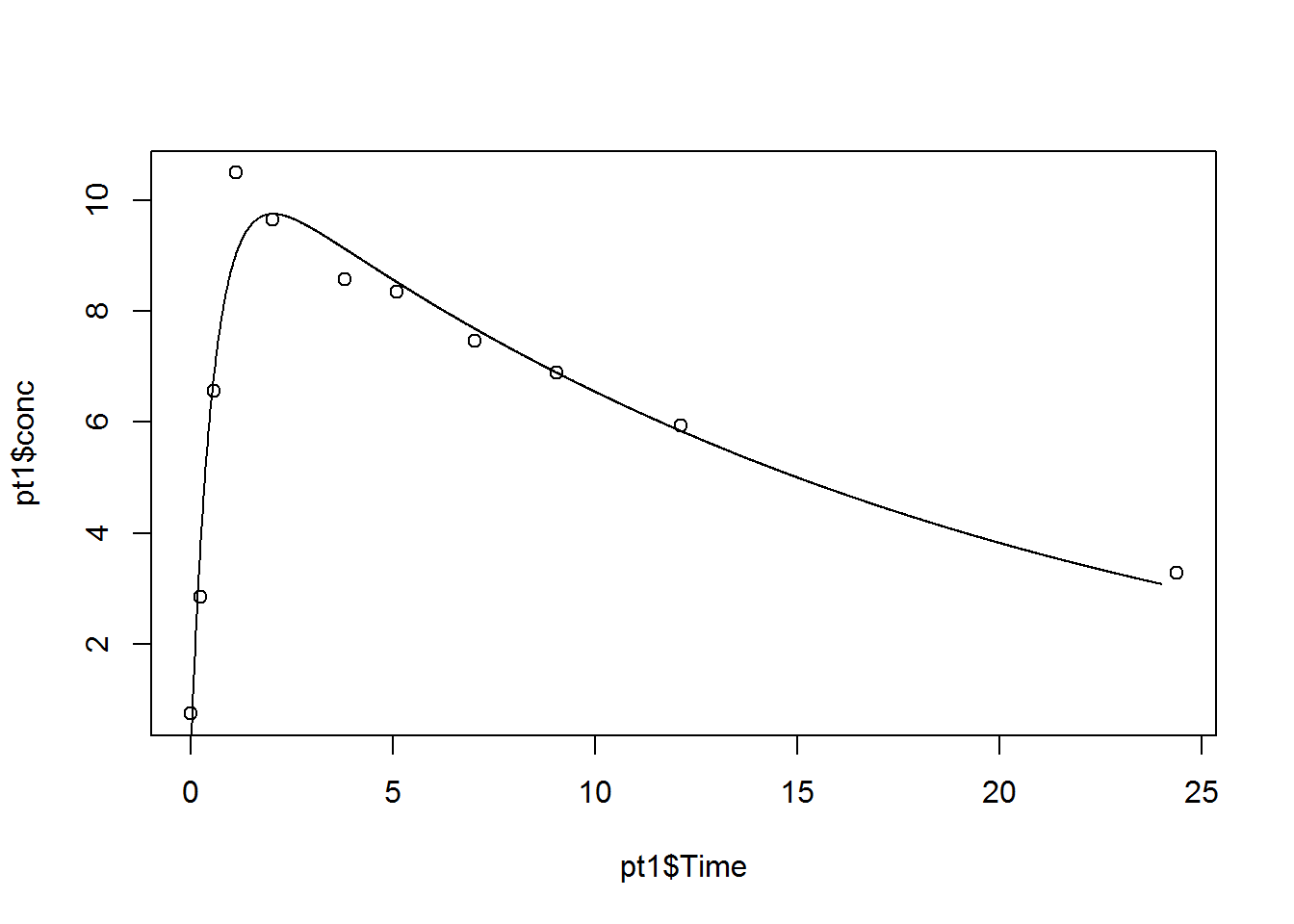``coef(mod1)``
``````##           a           b           c
## 11.22733466  0.05395463  1.77740848``````
``exp(coef(mod2))``
``````##        lke        lka        lcl
## 0.05395450 1.77741701 0.01992348``````
``````c2 <- coef(mod2)
a1 <- pt1\$Dose
a2 <- exp(c2["lke"]+c2["lka"]-c2["lcl"])
a3 <- exp(c2["lka"])-exp(c2["lke"])
print(a1*a2/a3)``````
``````##      lke
## 11.22732``````

Now let’s run this model separately for every patient in the data set.

``````mod3 <- list(NULL)
pt.list <- unique(as.character(Theoph\$Subject))
for (pt in pt.list) {
pt.sub <- Theoph[Theoph\$Subject==pt,]
mod3[[pt]] <- nls(conc~SSfol(Dose,Time,lke,lka,lcl),data=pt.sub)
plot(pt.sub\$Time,pt.sub\$conc,type="p",xlim=range(Theoph\$Time),ylim=range(Theoph\$conc))
title(paste("Patient",pt))
yp <- predict(mod3[[pt]],newdata=data.frame(Dose=rep(pt.sub\$Dose,length(t)),Time=t))
lines(t,yp)
}``````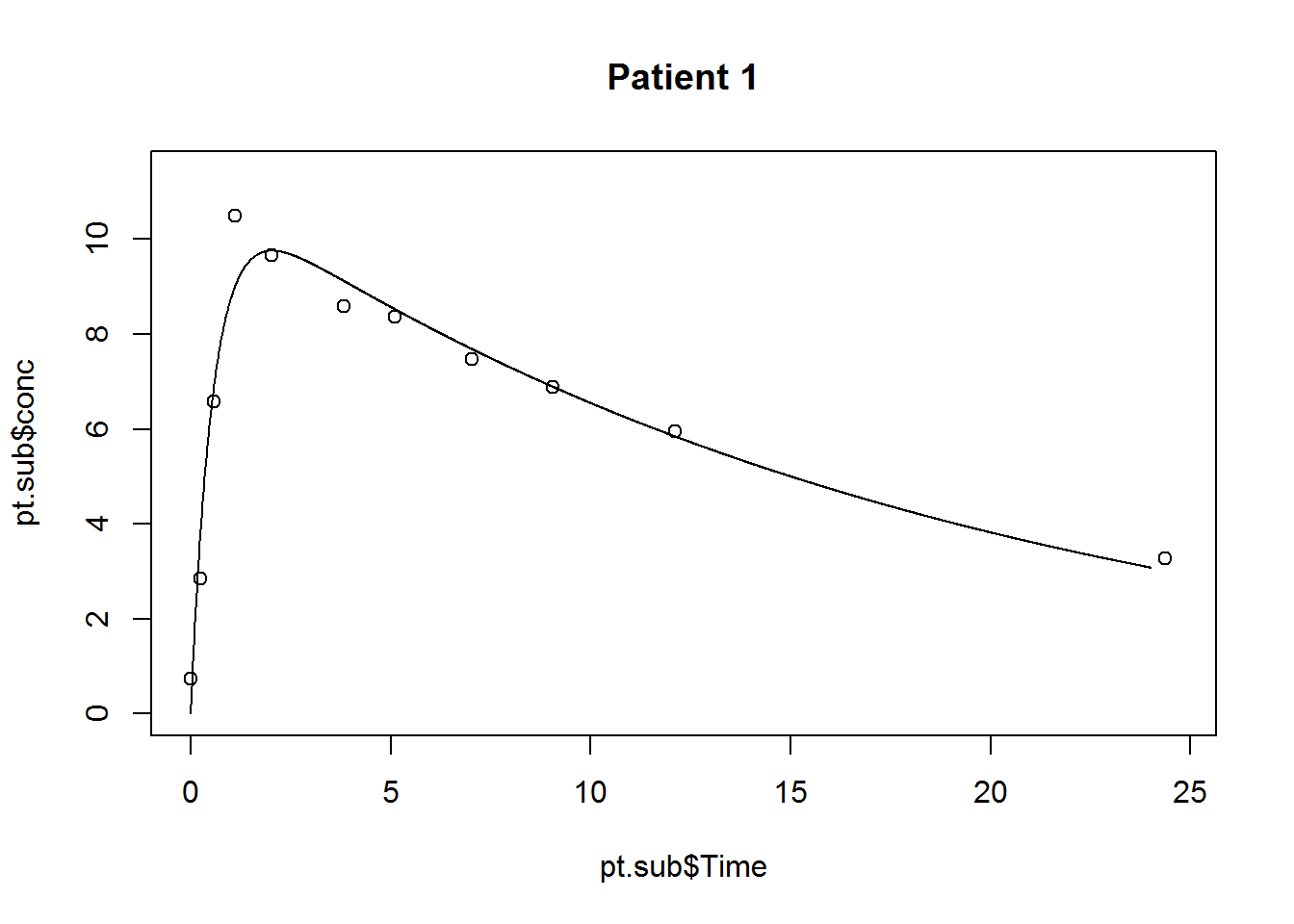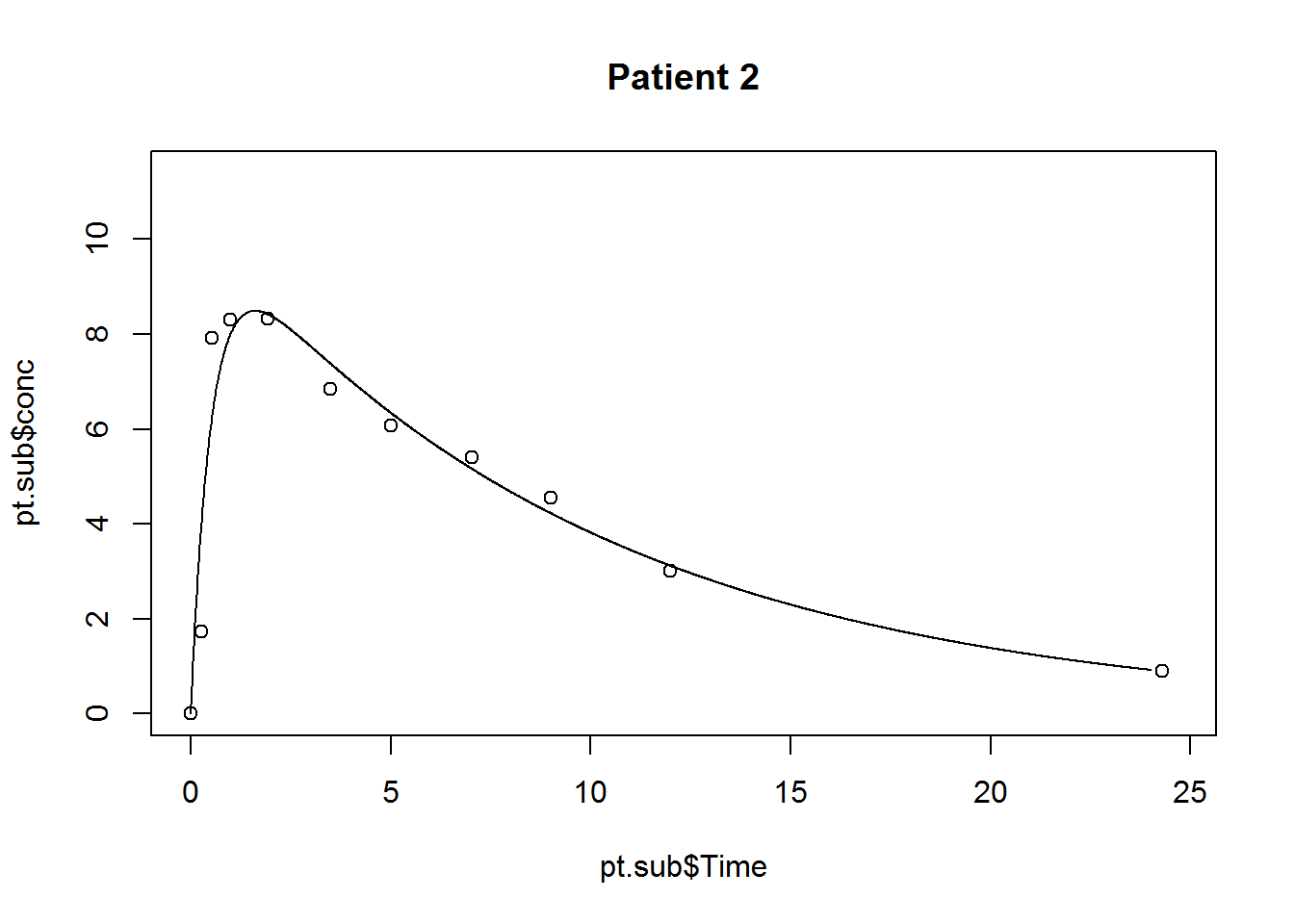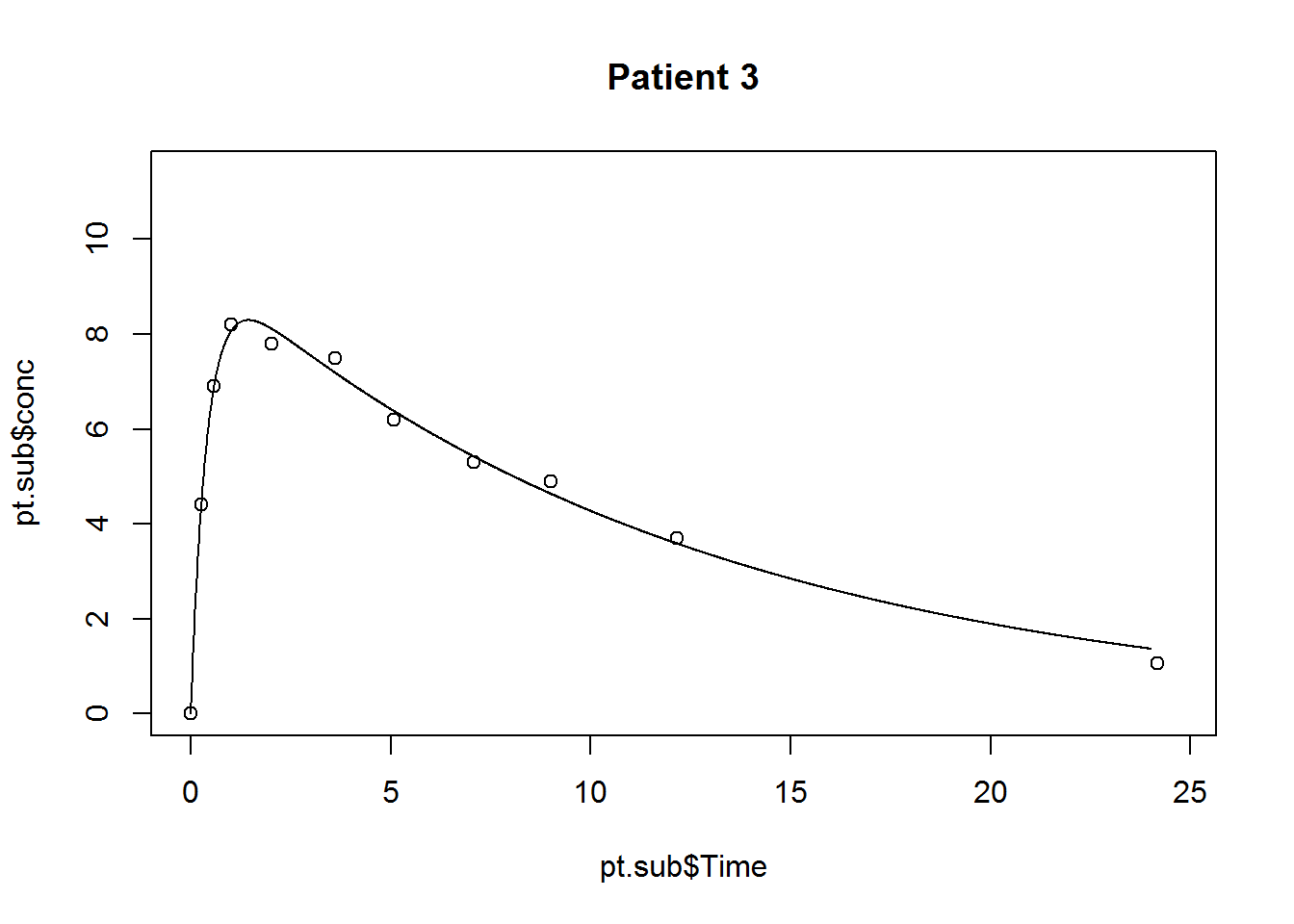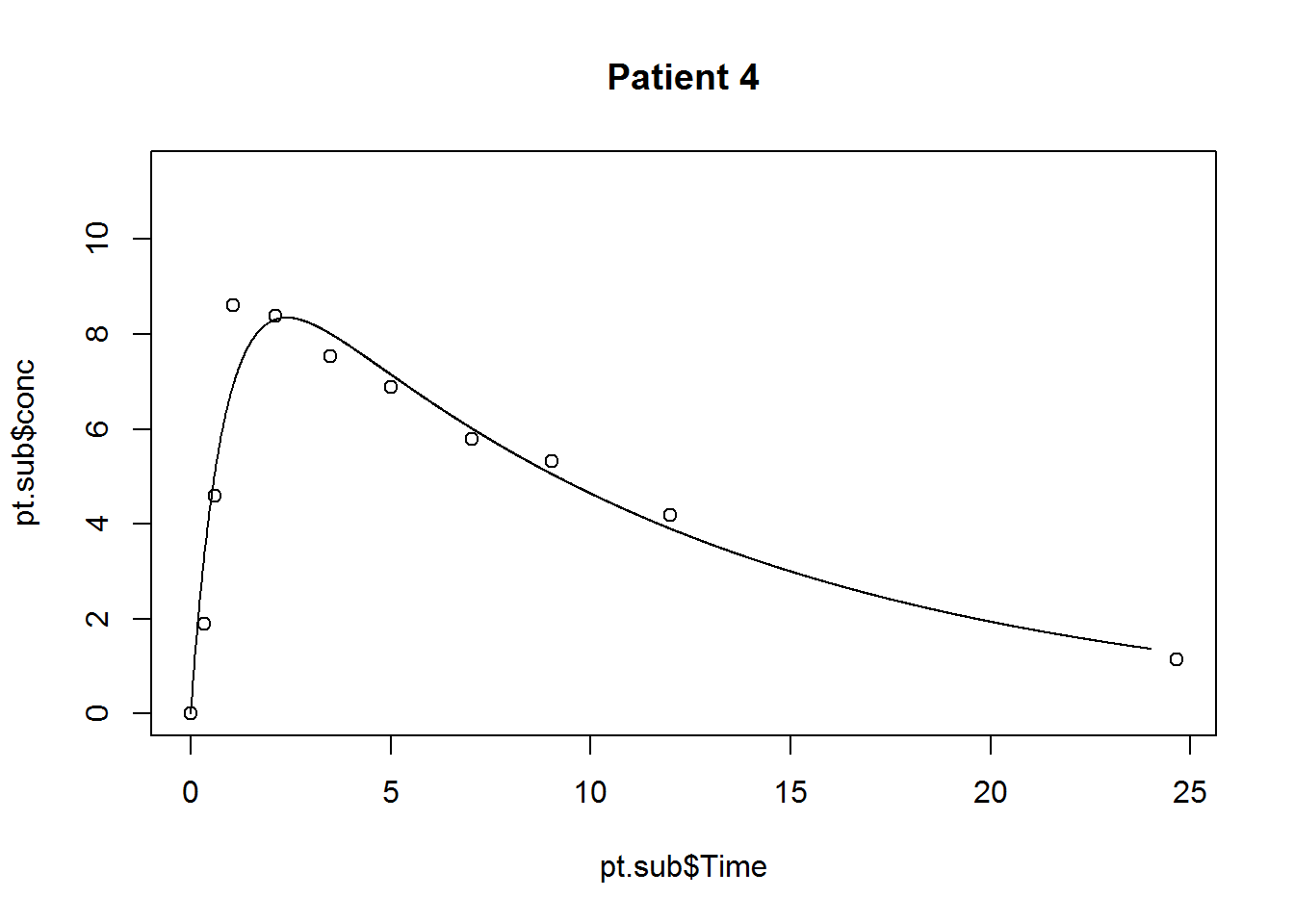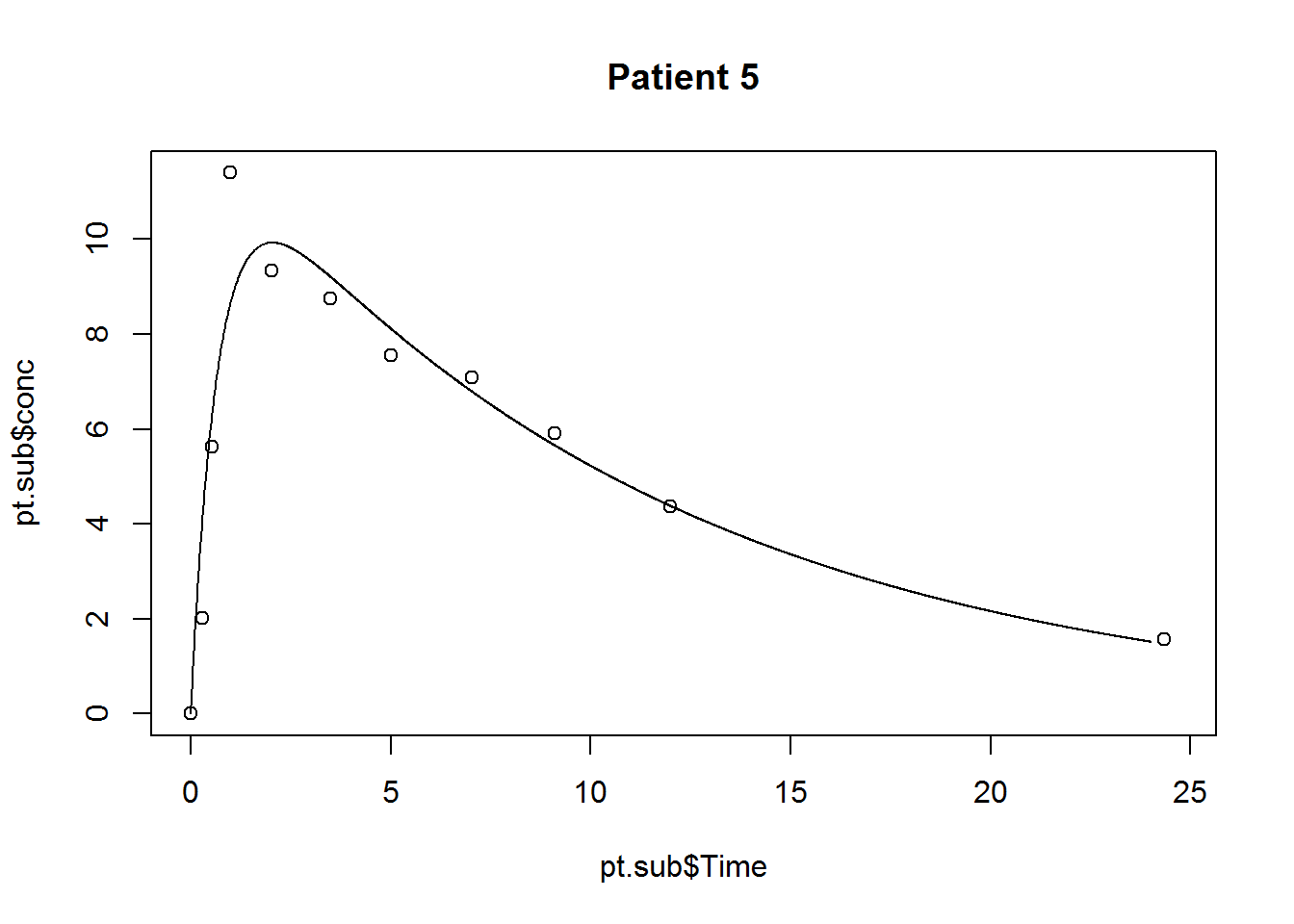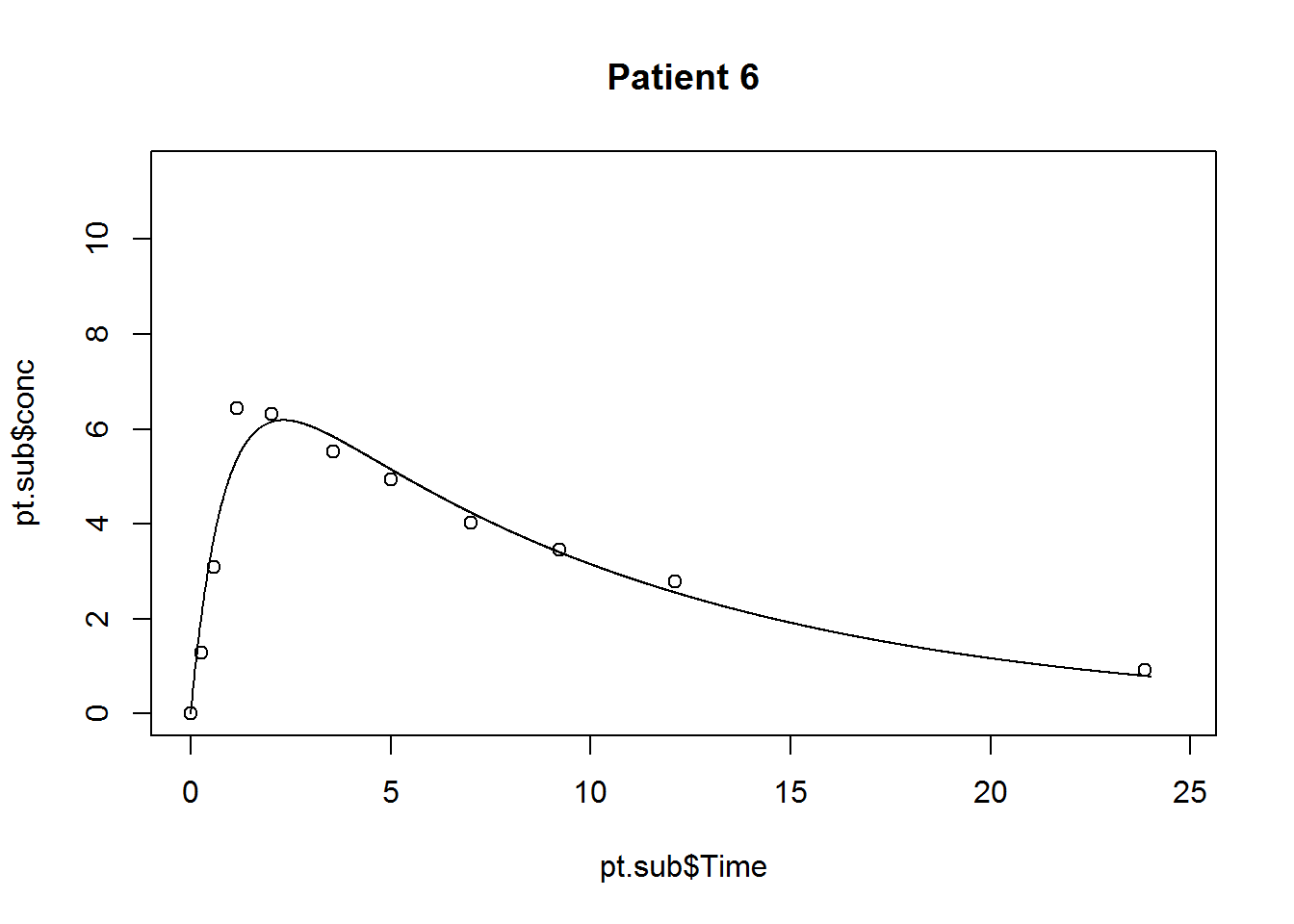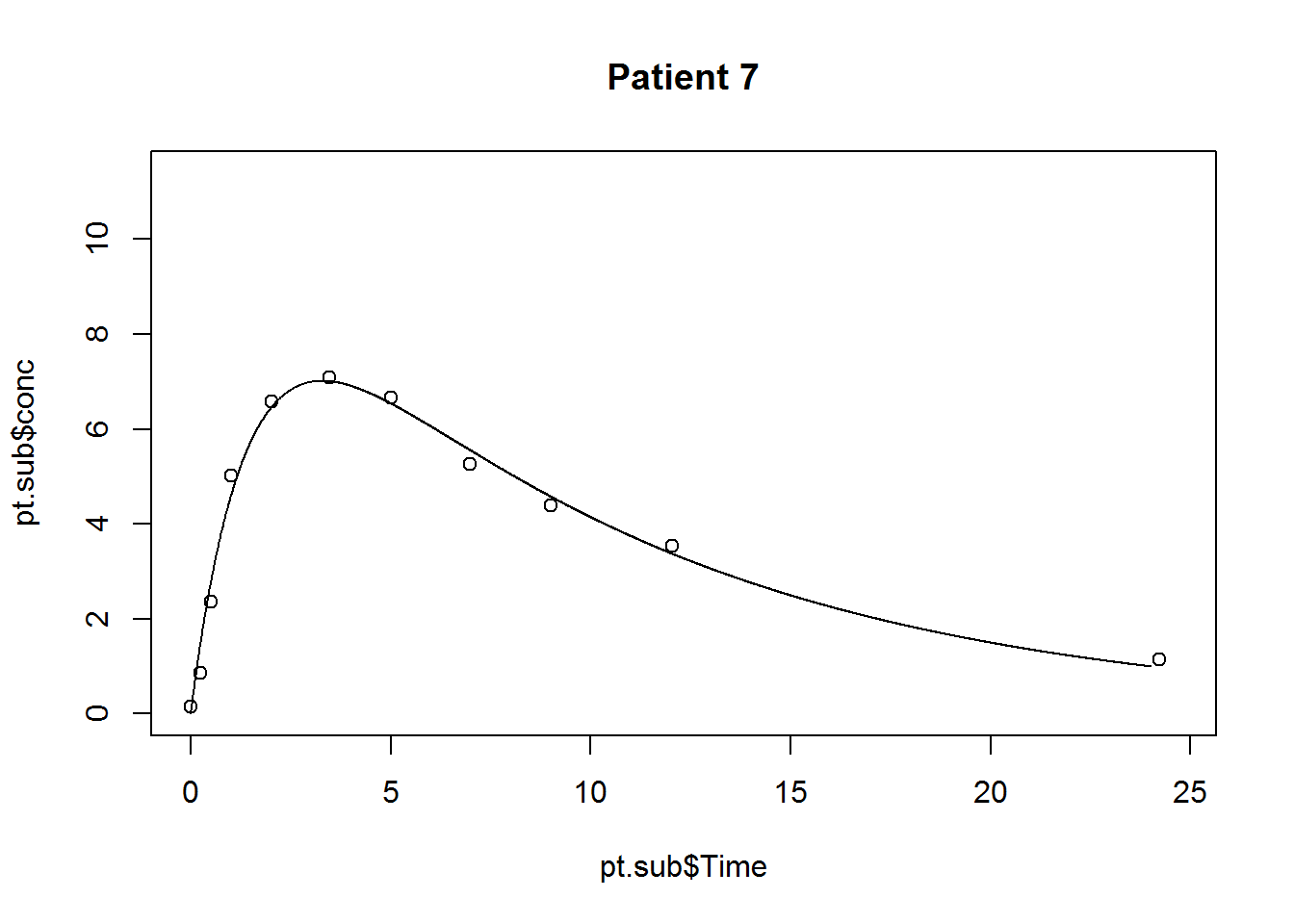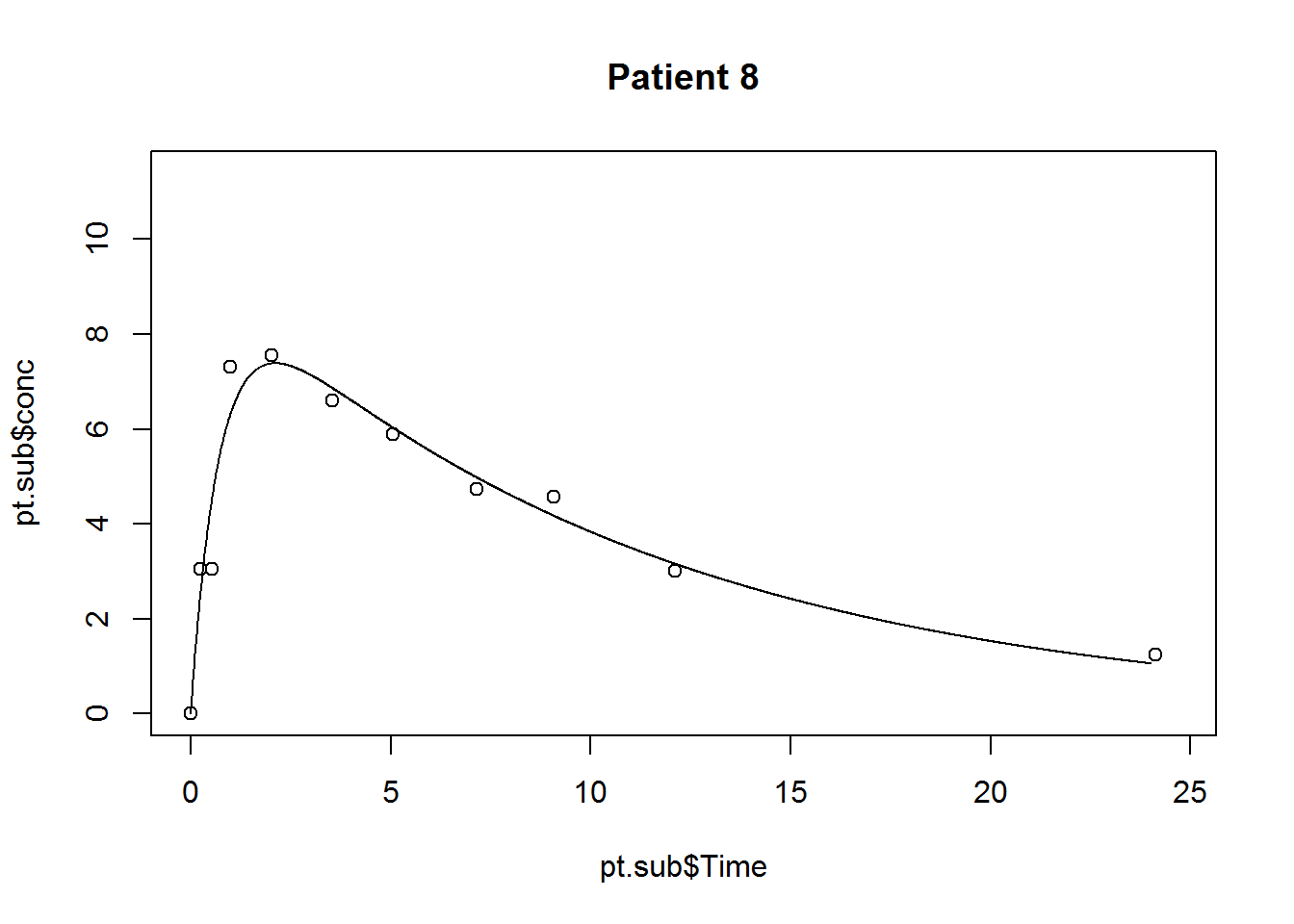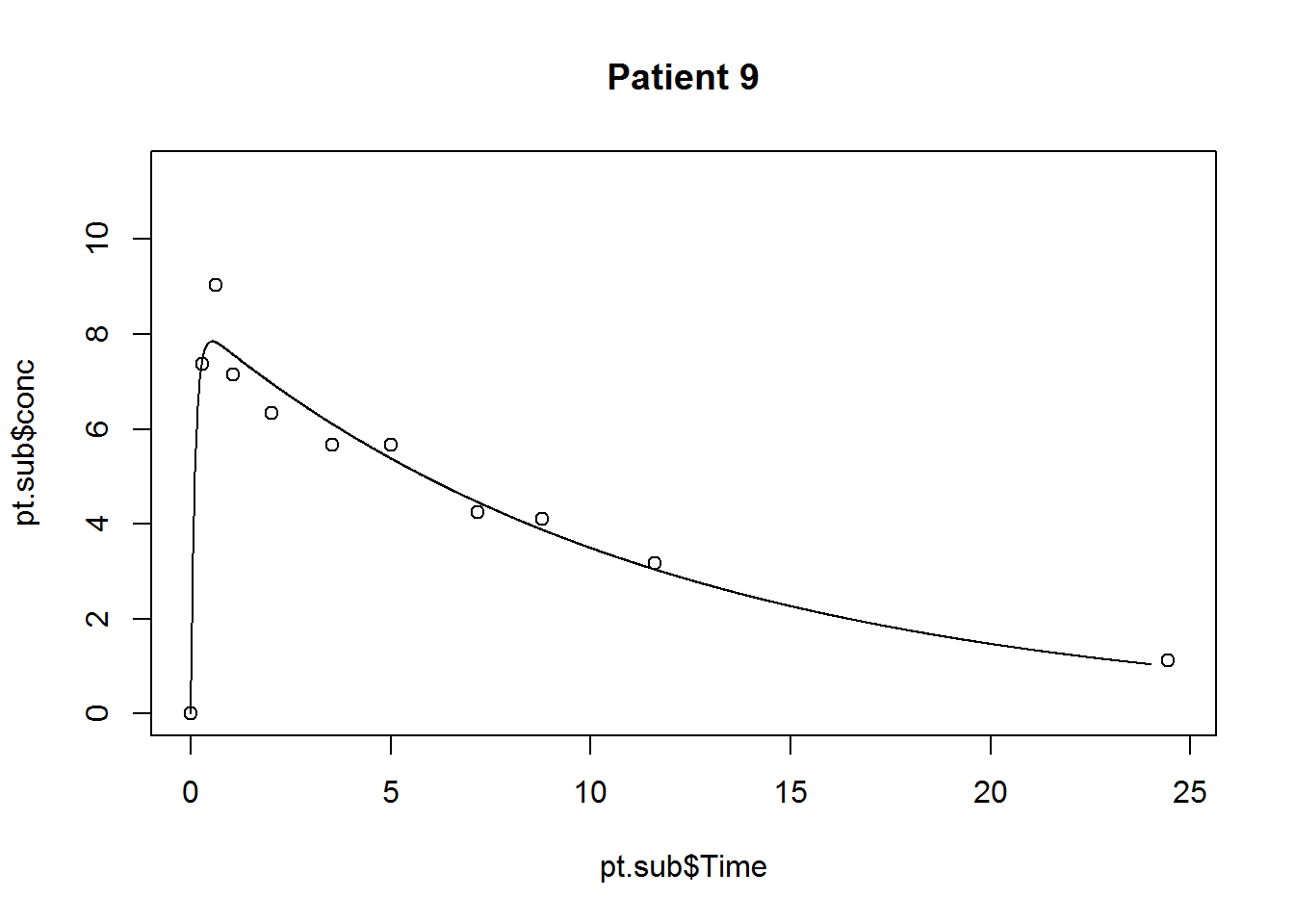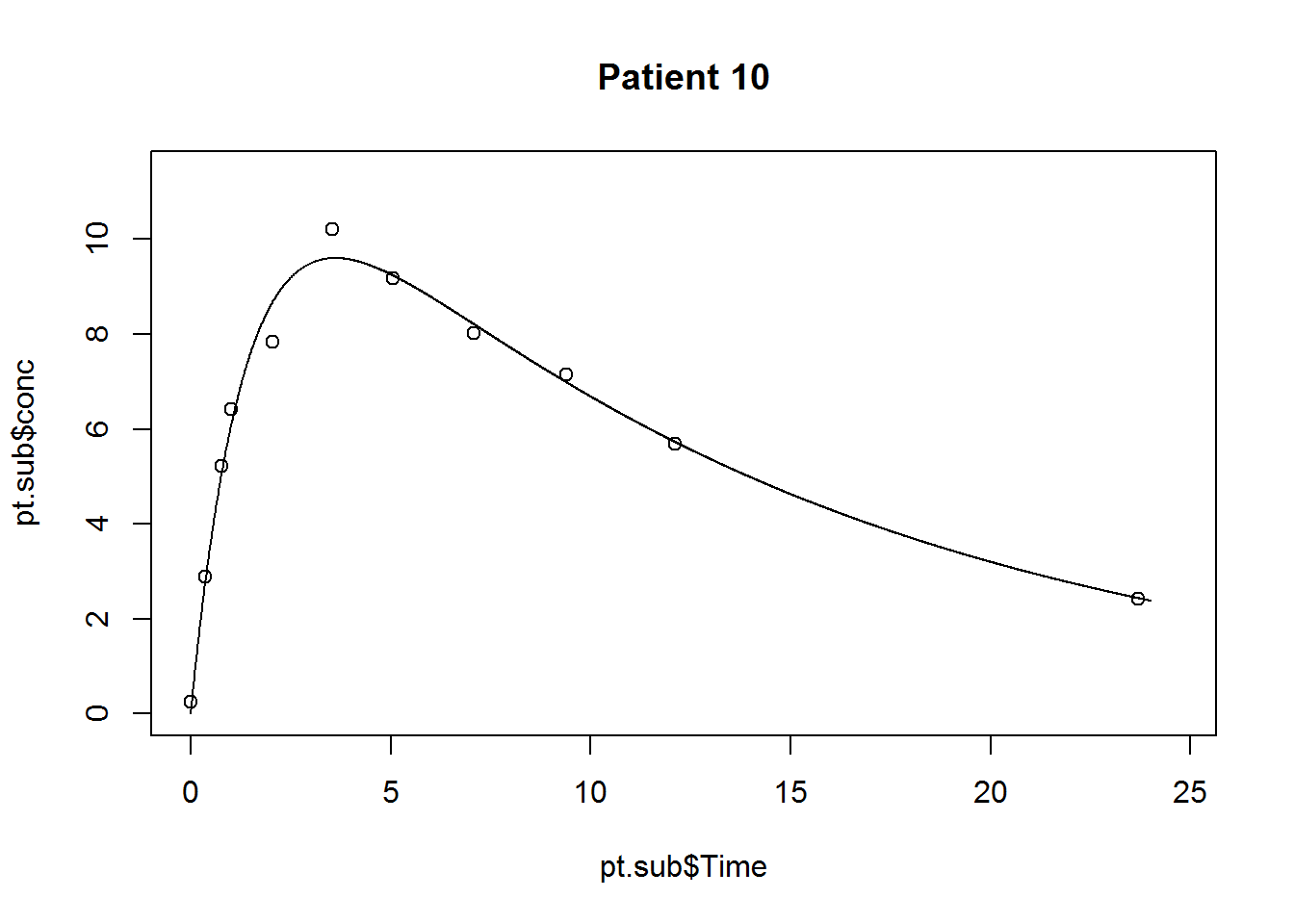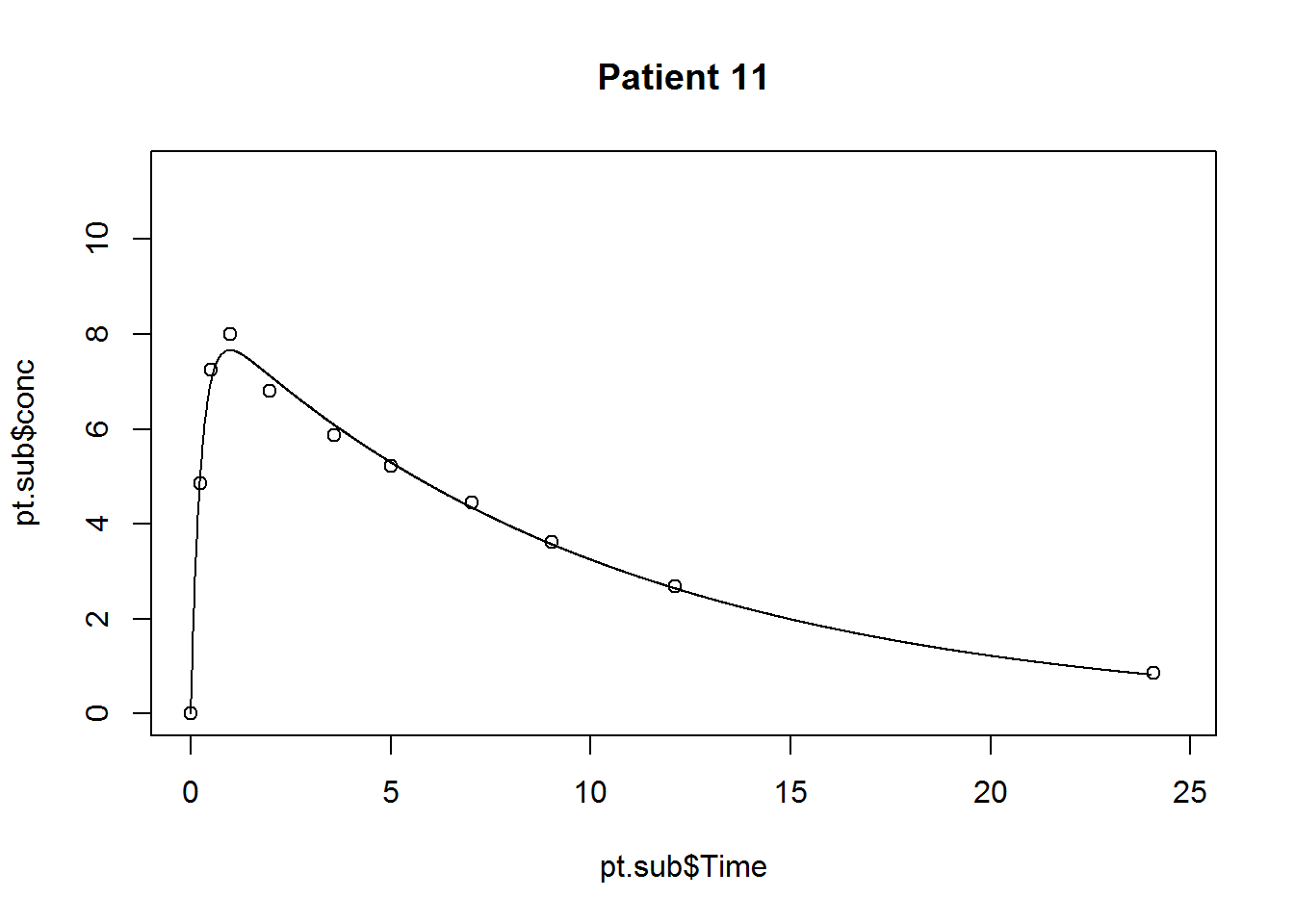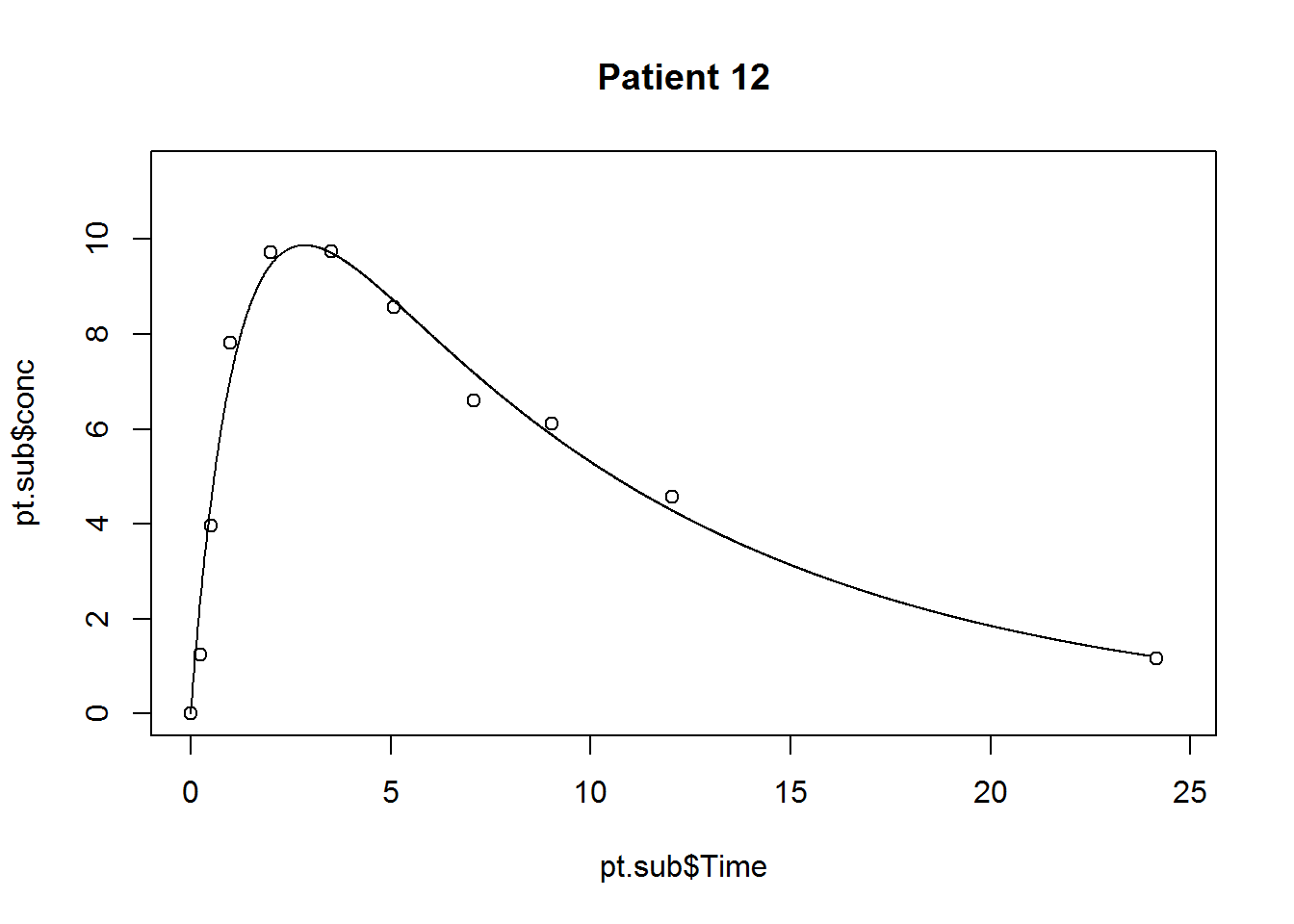``sapply(mod3,coef)``
``````## []
## NULL
##
## \$`1`
##        lke        lka        lcl
## -2.9196142  0.5751612 -3.9158566
##
## \$`2`
##        lke        lka        lcl
## -2.2861083  0.6640568 -3.1063169
##
## \$`3`
##        lke        lka        lcl
## -2.5080732  0.8975422 -3.2299646
##
## \$`4`
##        lke        lka        lcl
## -2.4364940  0.1582638 -3.2860869
##
## \$`5`
##        lke        lka        lcl
## -2.4254859  0.3862853 -3.1326003
##
## \$`6`
##        lke        lka        lcl
## -2.3073316  0.1516234 -2.9732417
##
## \$`7`
##        lke        lka        lcl
## -2.2803698 -0.3860511 -2.9643353
##
## \$`8`
##        lke        lka        lcl
## -2.3864369  0.3188339 -3.0691110
##
## \$`9`
##       lke       lka       lcl
## -2.446088  2.182188 -3.420774
##
## \$`10`
##        lke        lka        lcl
## -2.6041476 -0.3631216 -3.4282705
##
## \$`11`
##       lke       lka       lcl
## -2.321530  1.347824 -2.860397
##
## \$`12`
##        lke        lka        lcl
## -2.2483257 -0.1828442 -3.1701582``````

## Save results for later use

``save.image("nonlinear.RData")``